## Monday, June 19, 2017

2014
MATHEMATICS
Full Marks: 100
Pass Marks: 30

Time: 3 hours
The figures in the margin indicate full marks for the questions

1. Answer the following questions: 1x10=10
1. Is multiplication a binary operation on the set {0}
2. Ifwrite down the value of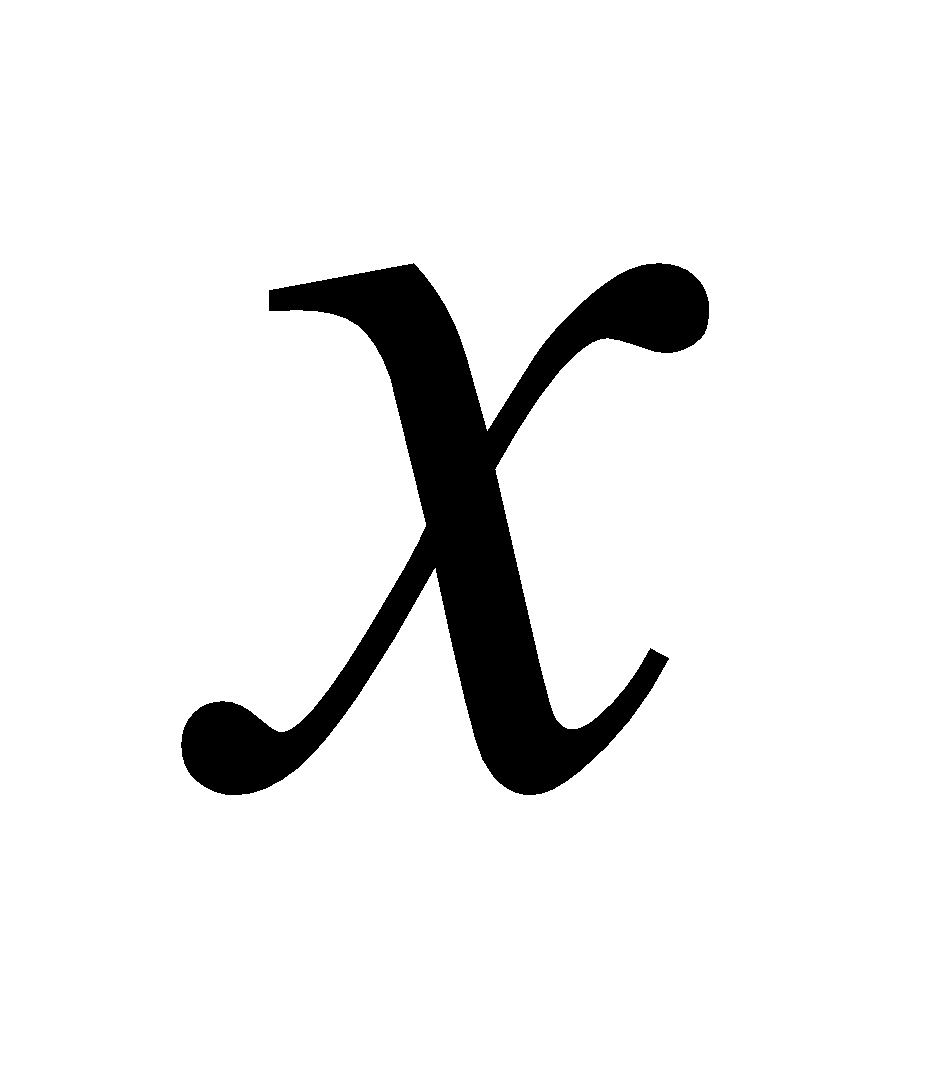.
3. If A be a square matrix of order 2 whose determinant is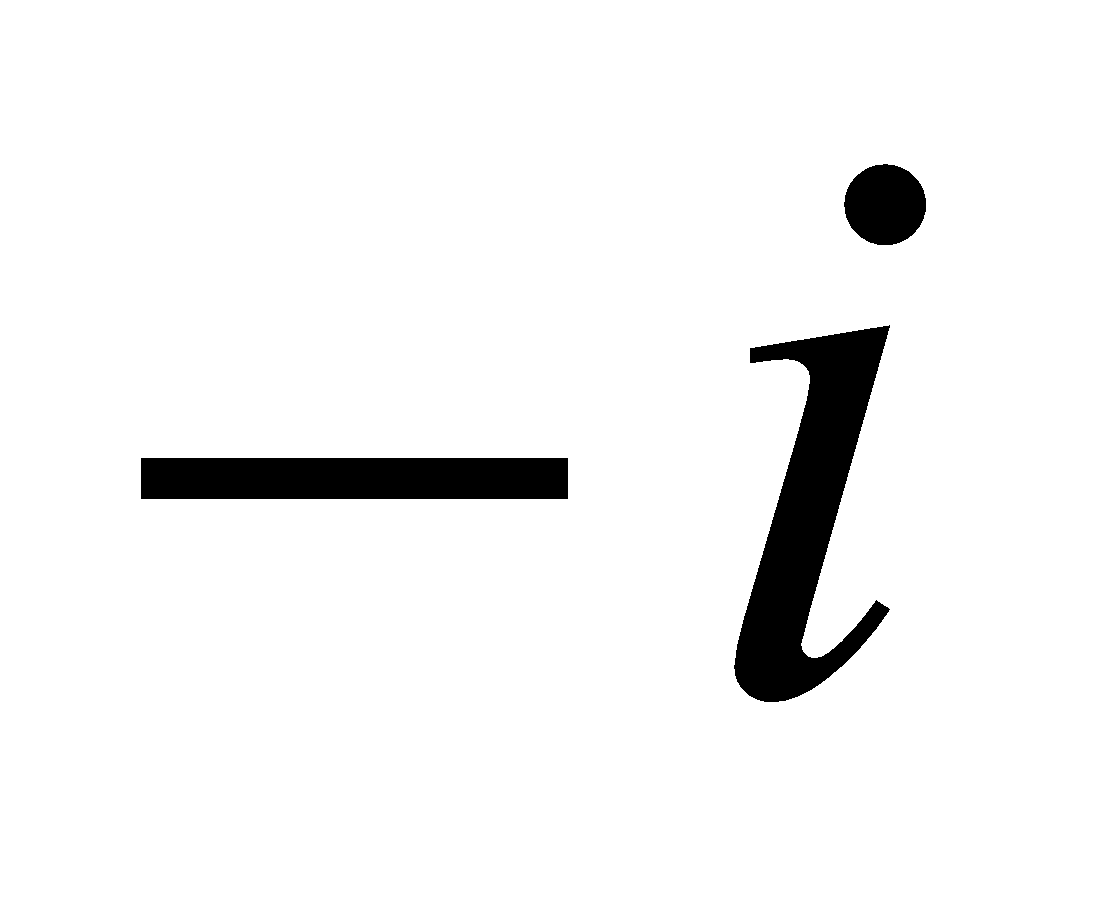, find the value of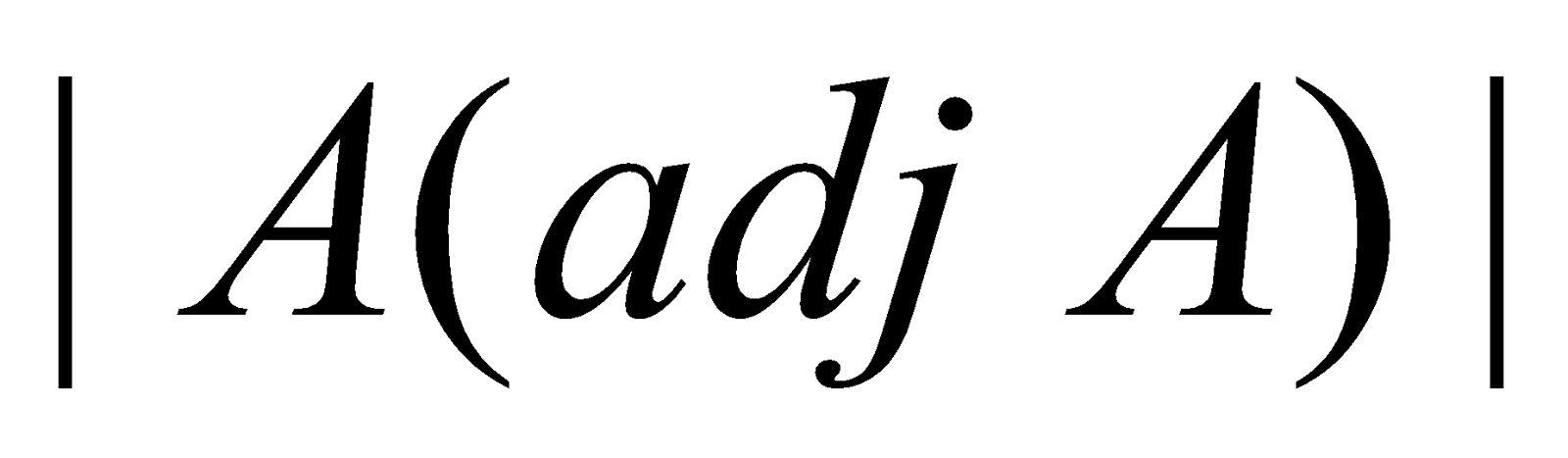4. What is the number of all possible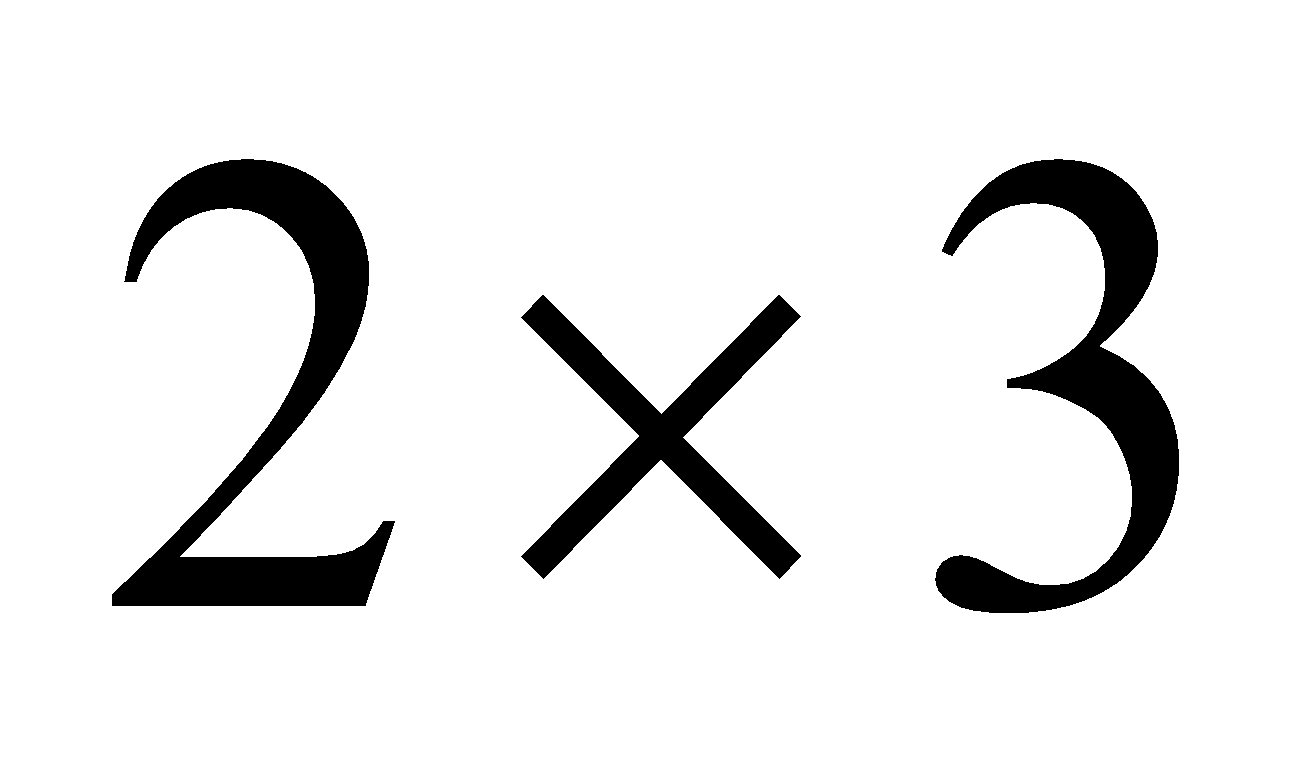matrices with entries 0 or 1?
5. If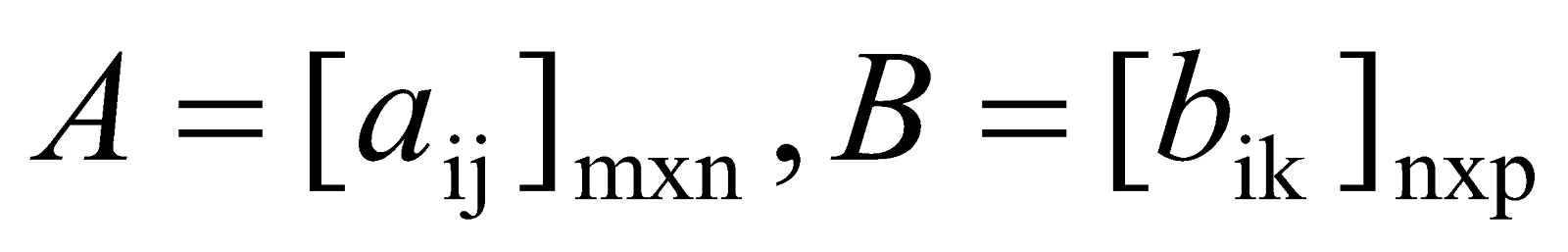be two matrices and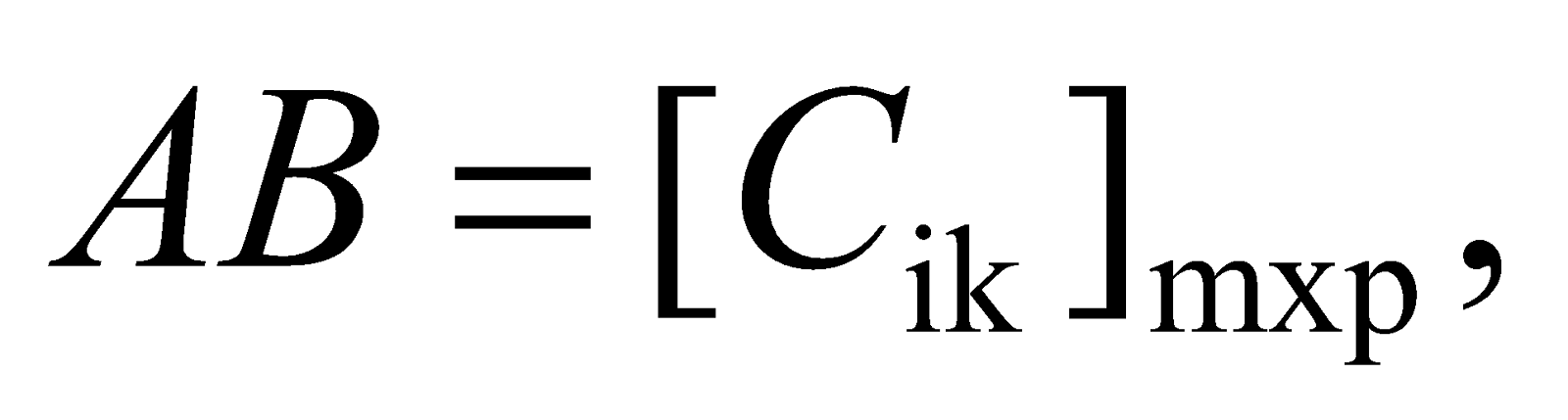then what is the relation among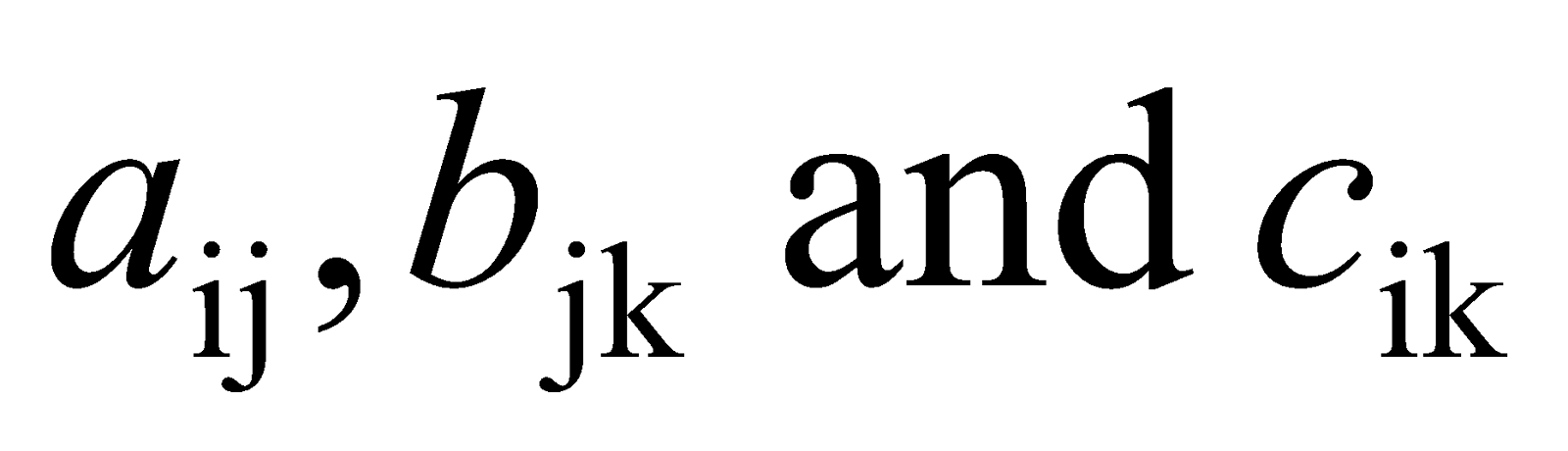?
6. What is the value of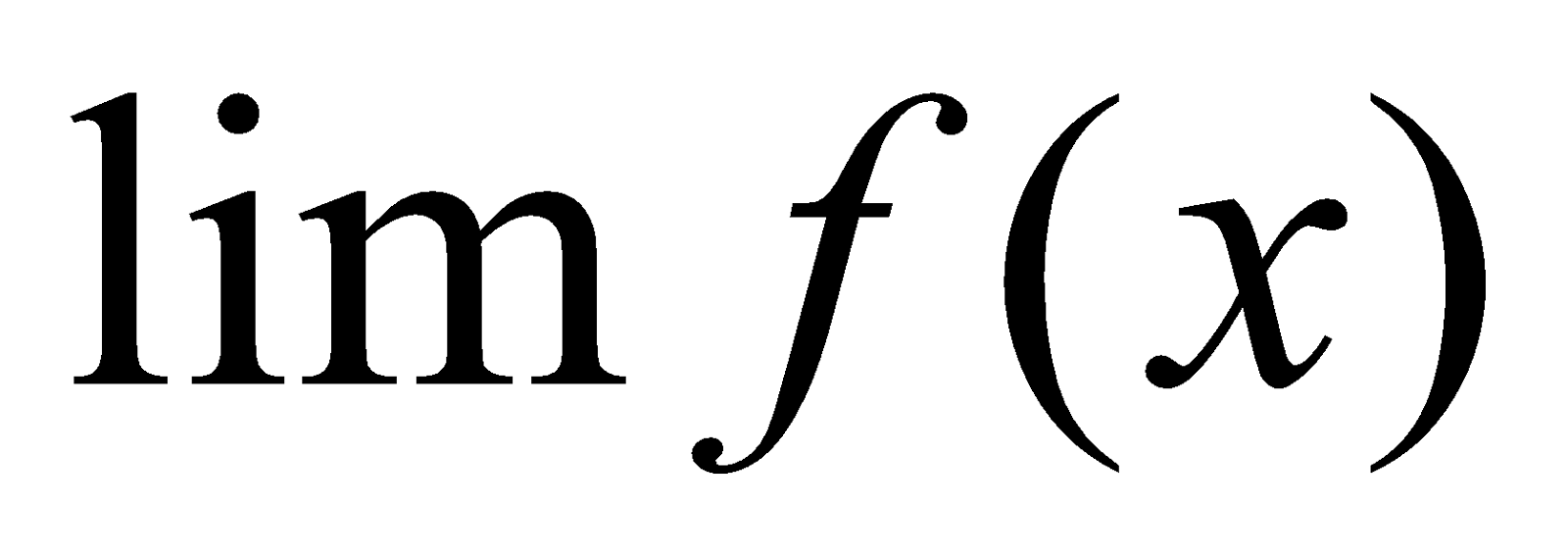if a function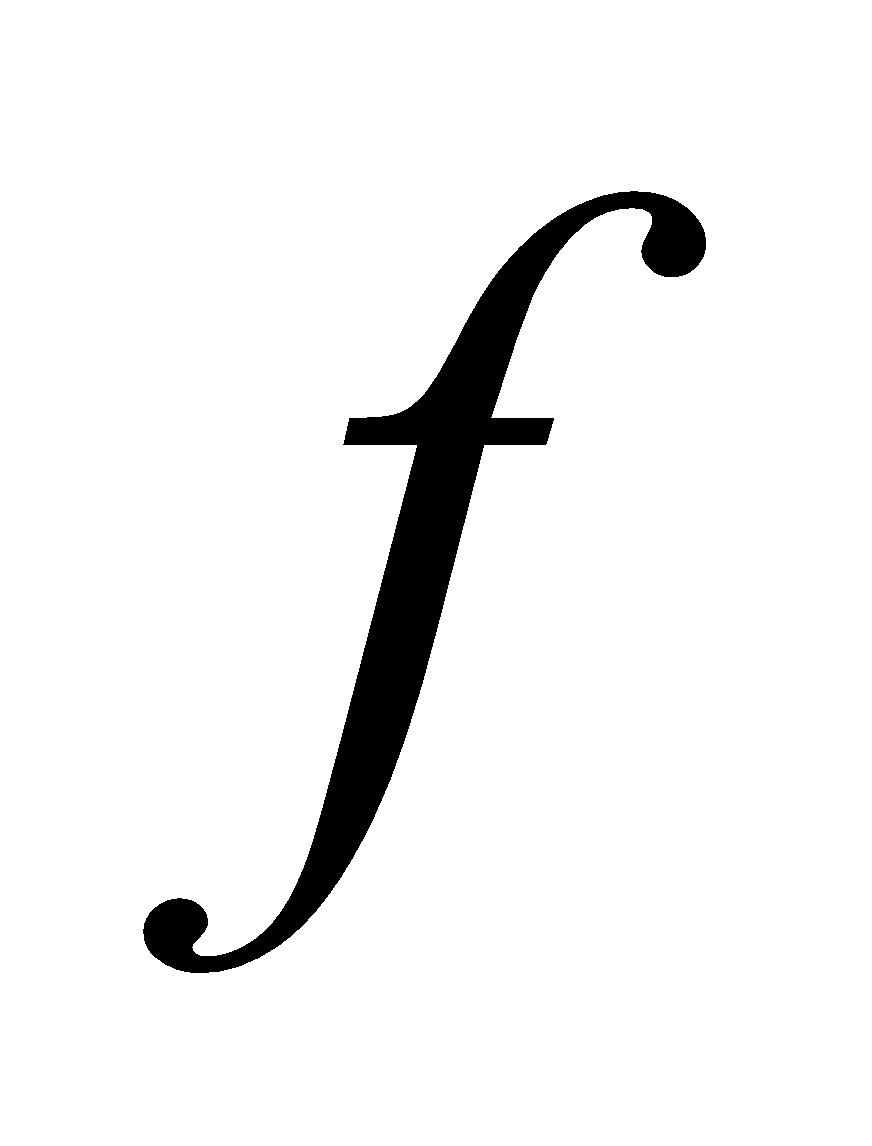is continuous at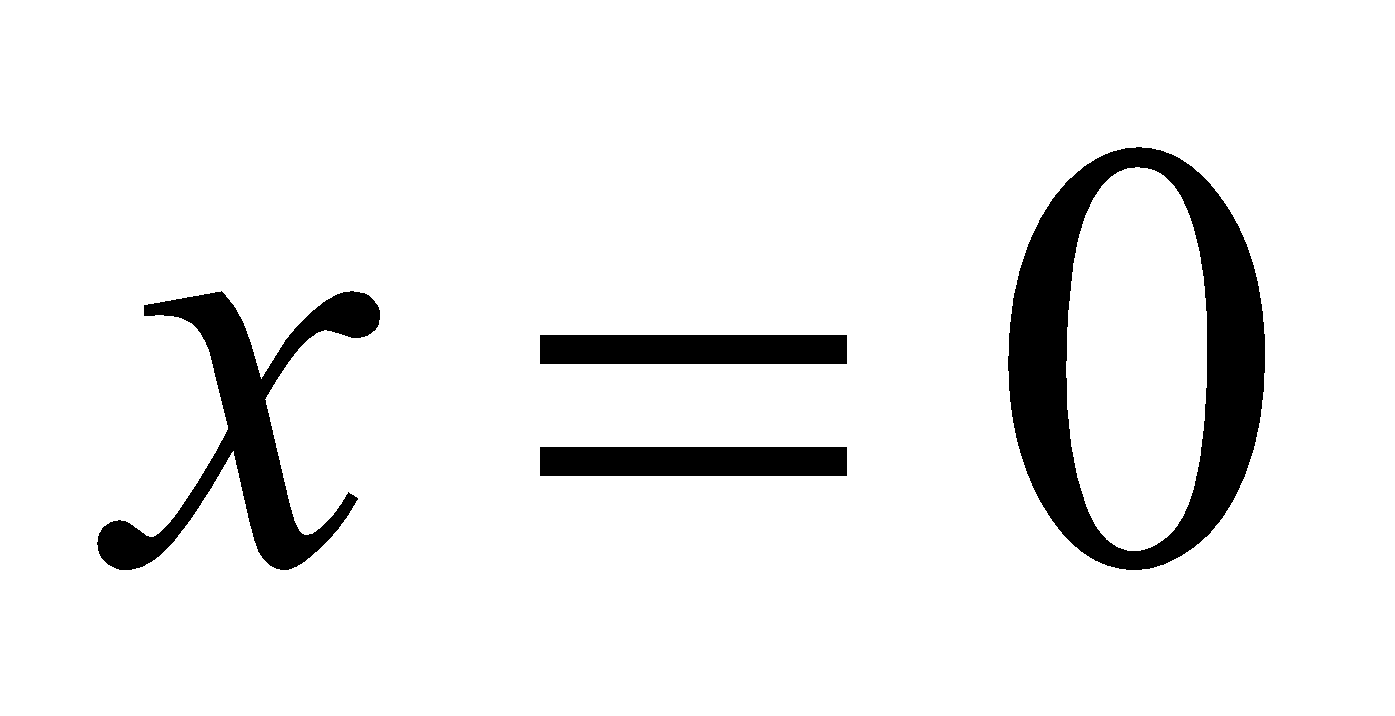and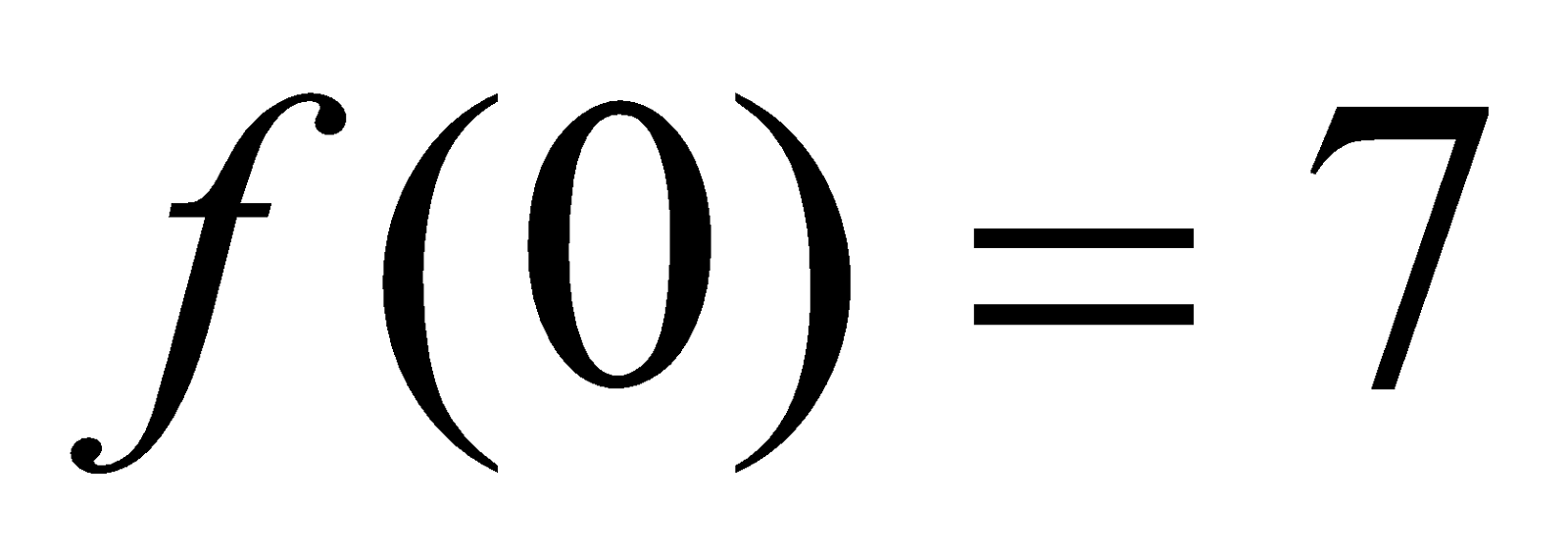?
7. State whether it is true or false: “The derivative of an even function is always an even function”.
8. If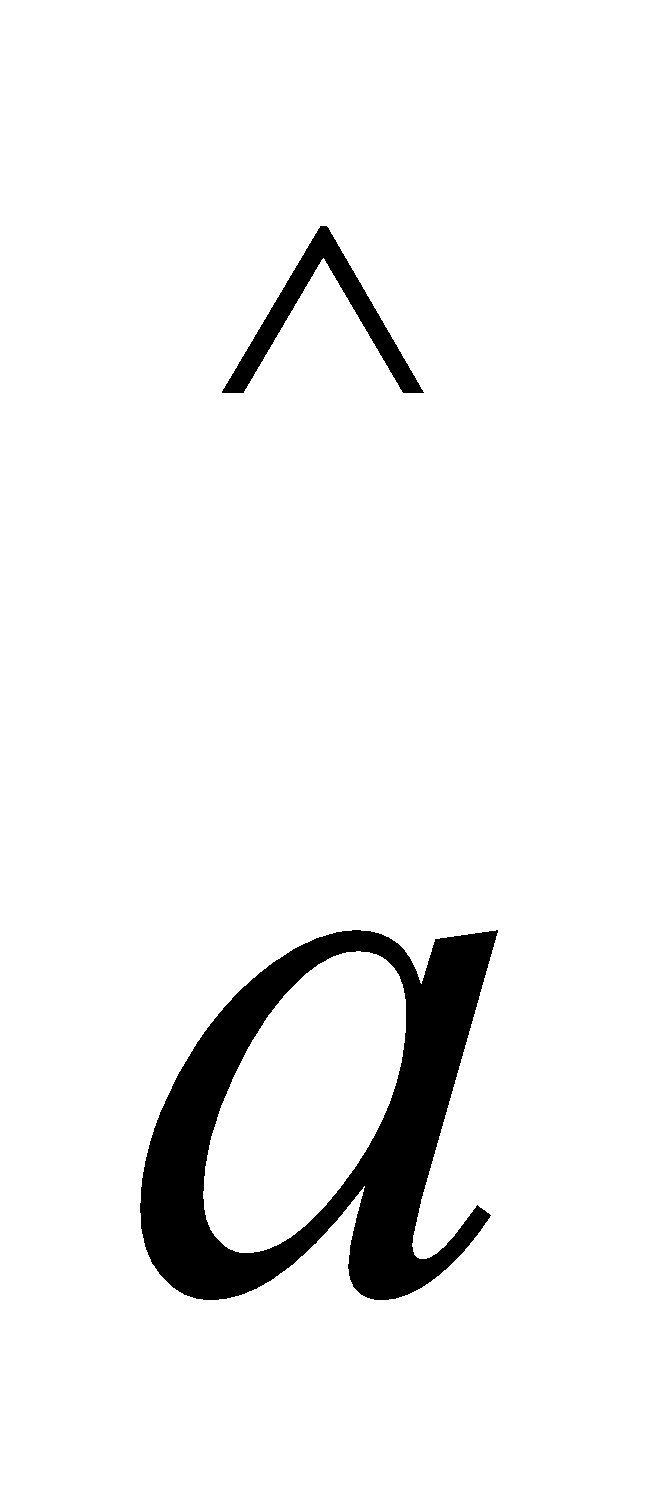and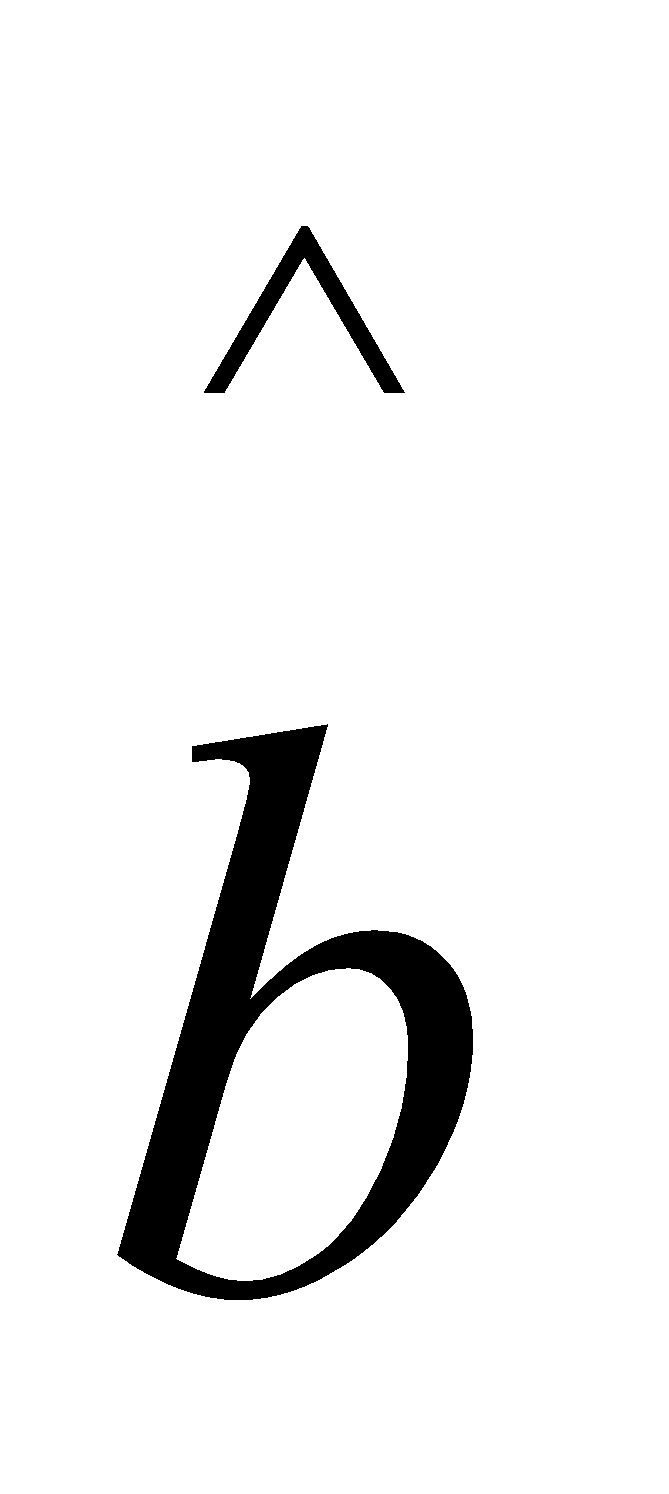are two mutually perpendicular unit vectors, what is the value of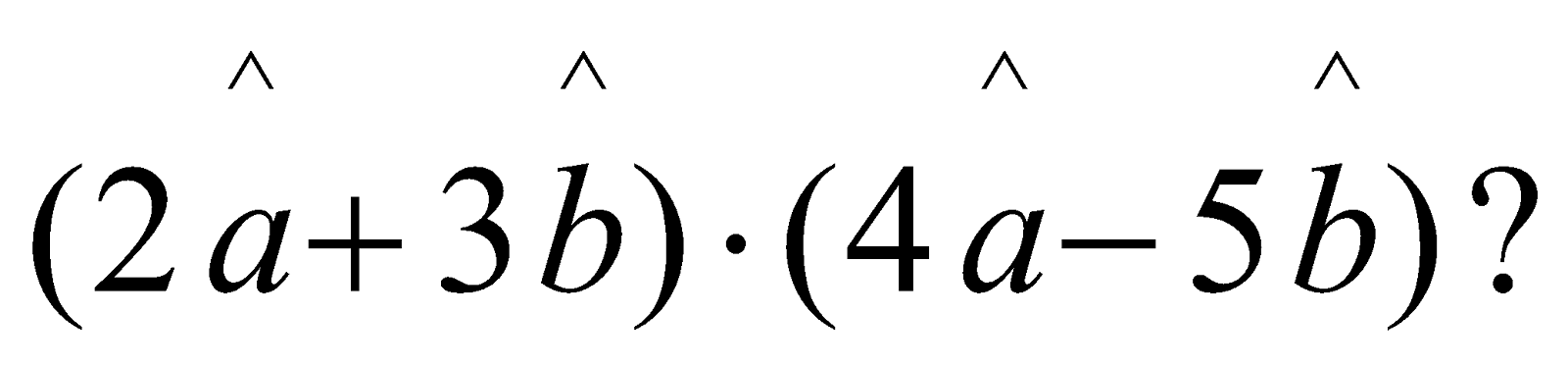9. What is the general equation of a plane parallel to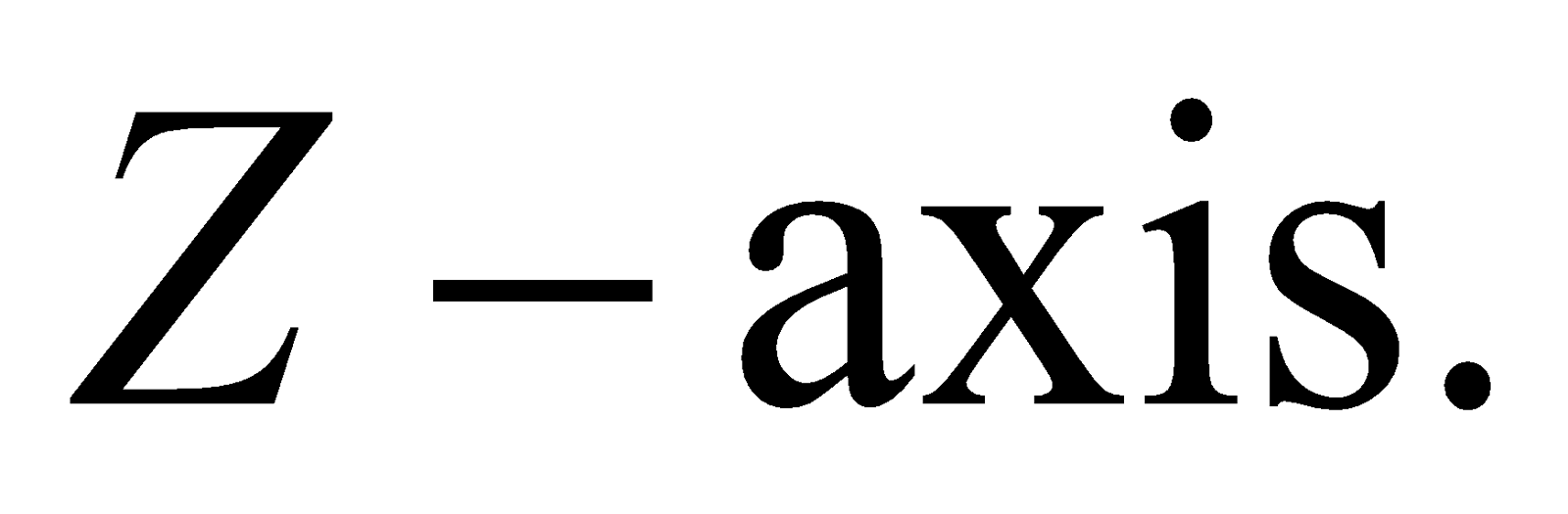10. Let l, m, n be the direction cosines of the linewhere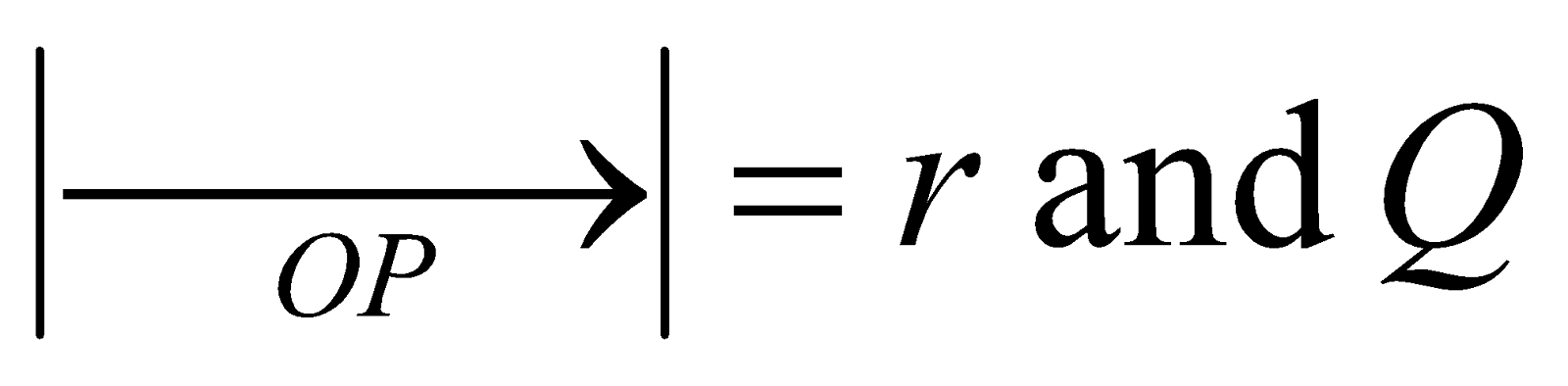be any point on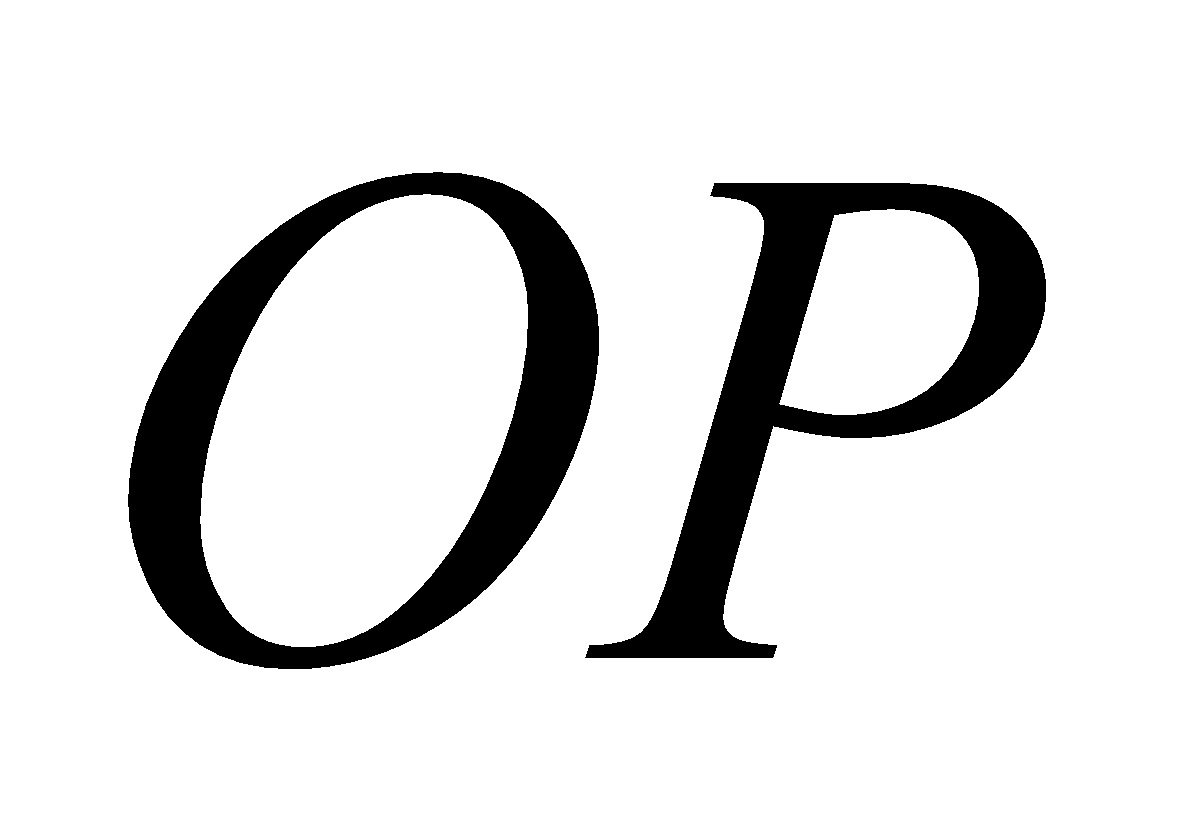where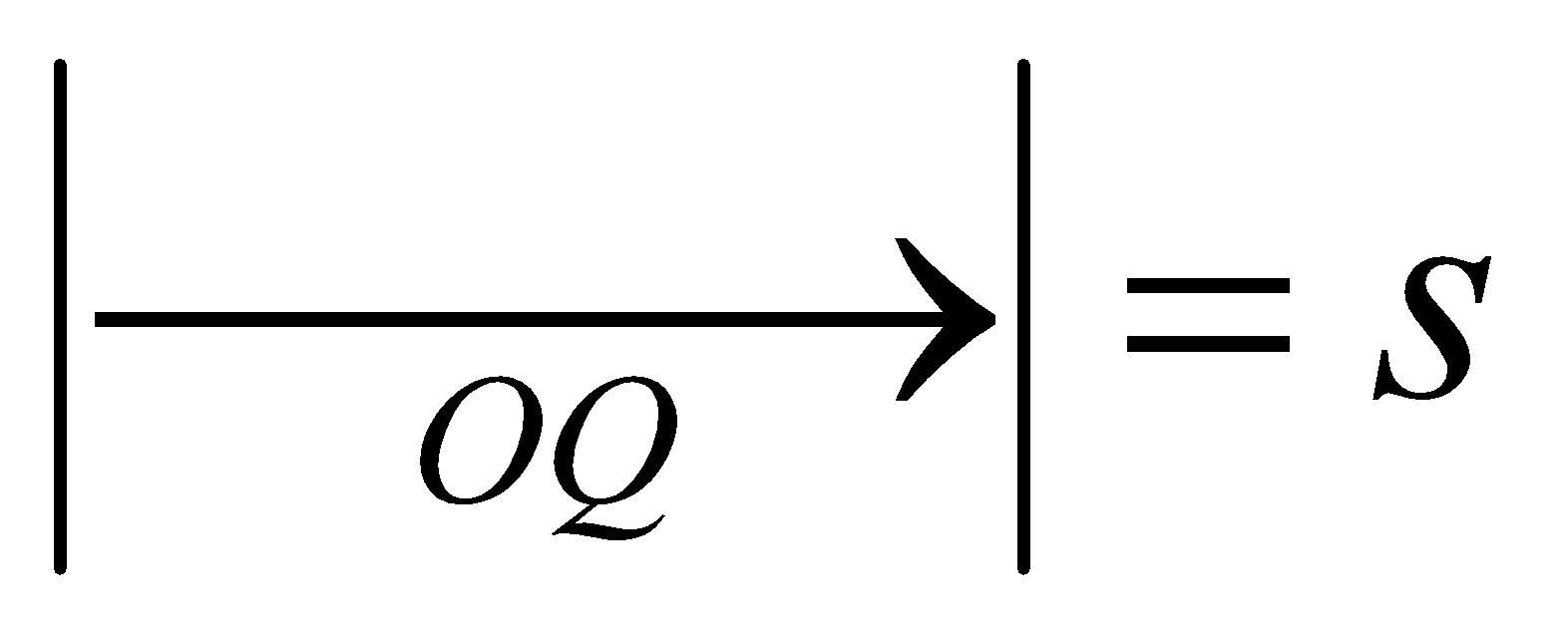. What is the co-ordinate of the mid-point of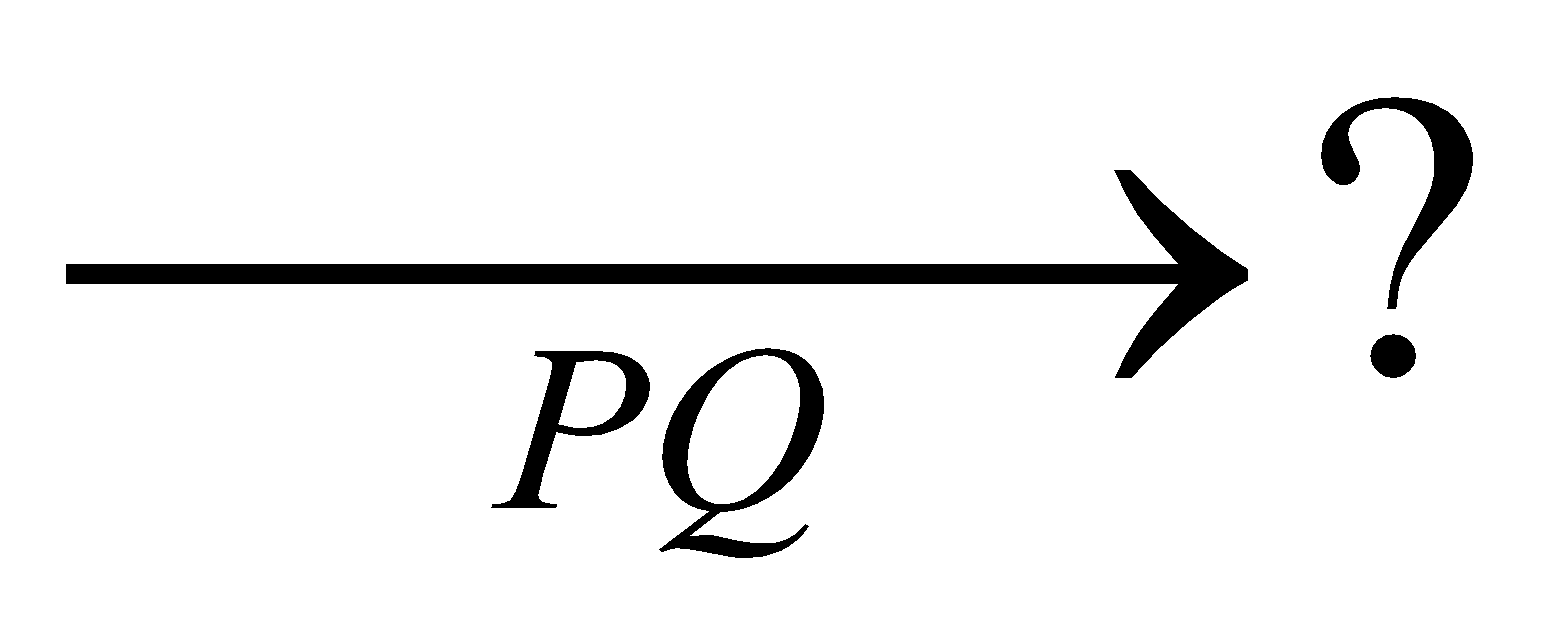2. Let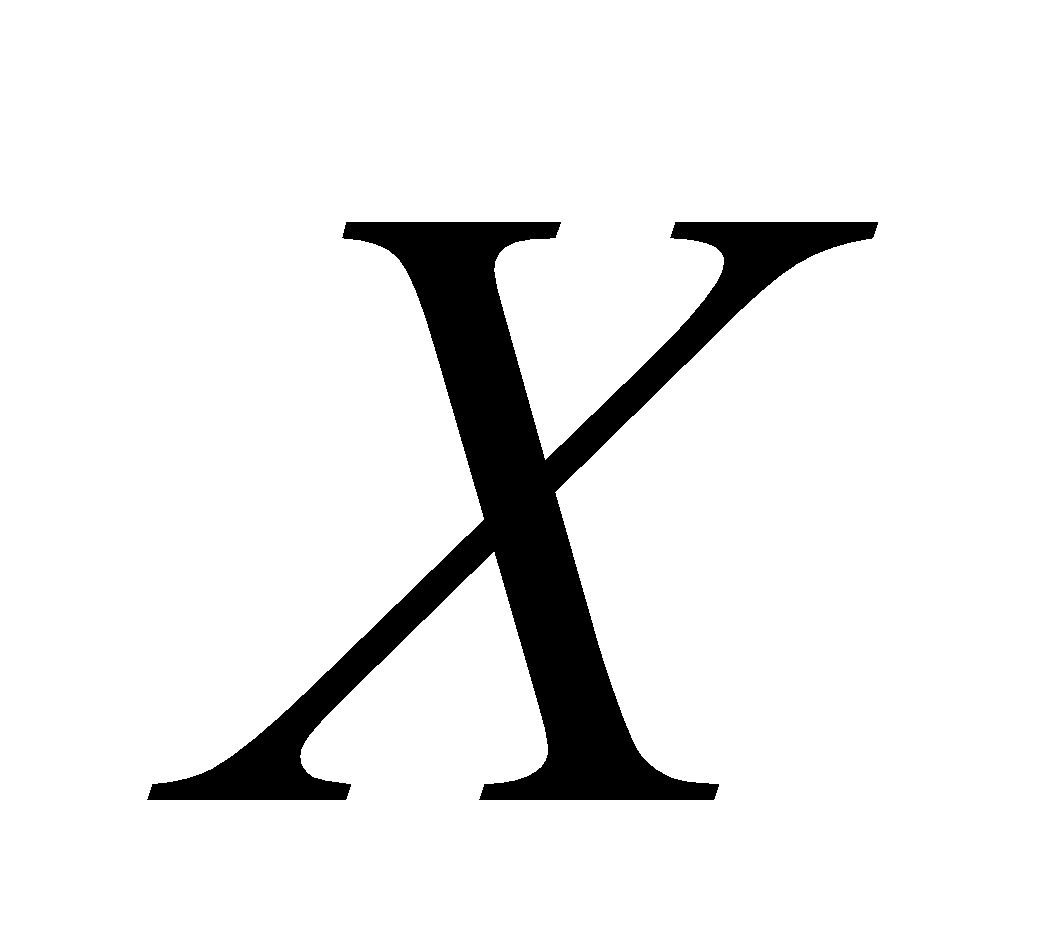be a non-empty set and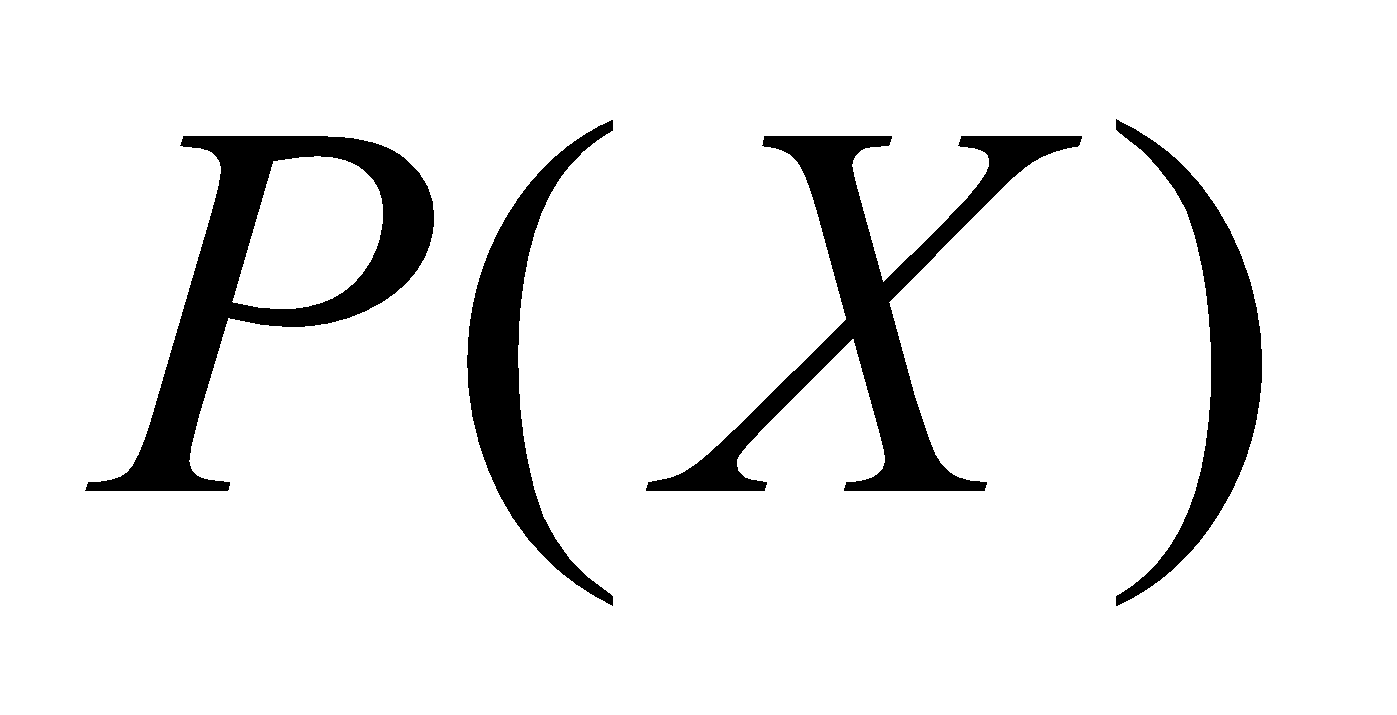be the power set of. Consider the binary operation * ondefined by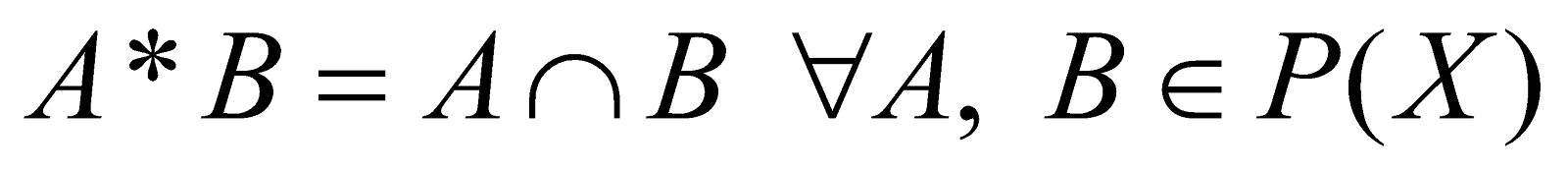. Show thatis the identity element as well as the only invertible element inw.r.t * 4
3. Solve the following equation for: 4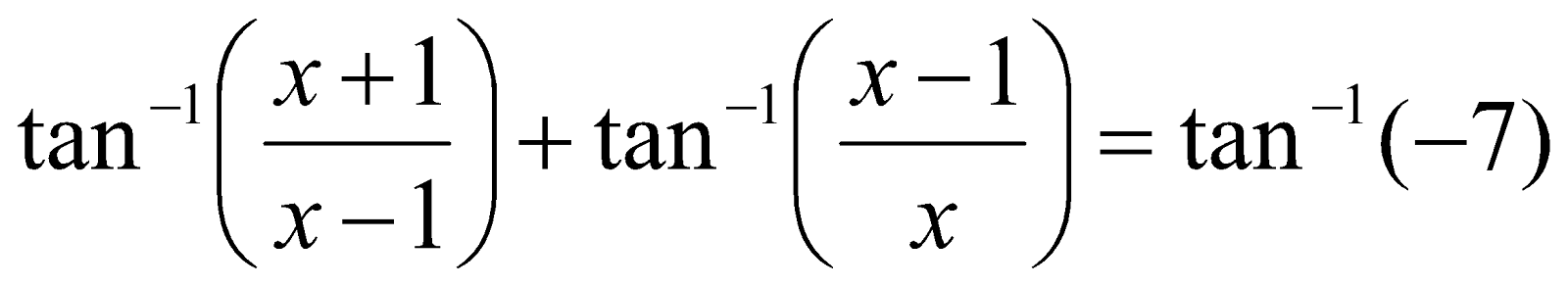Or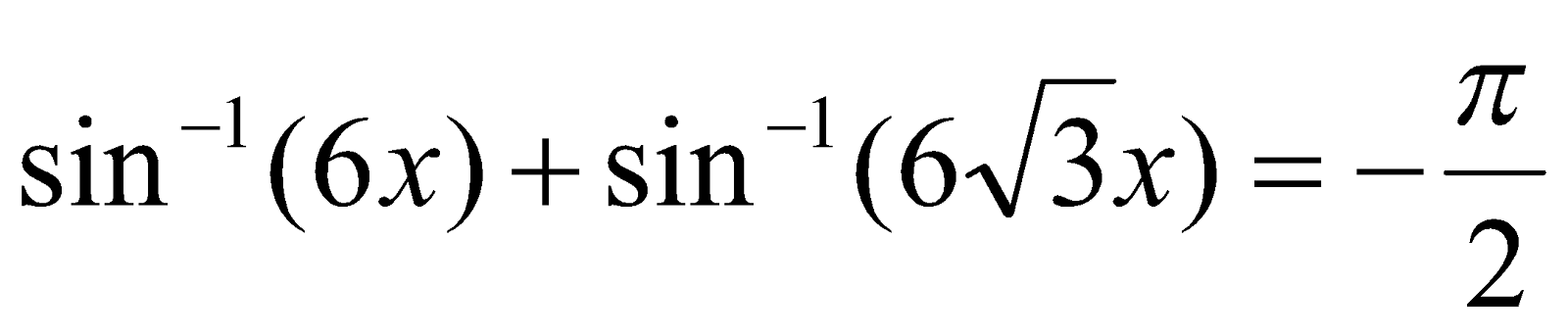4. Answer the following questions: 2+2=4
1. If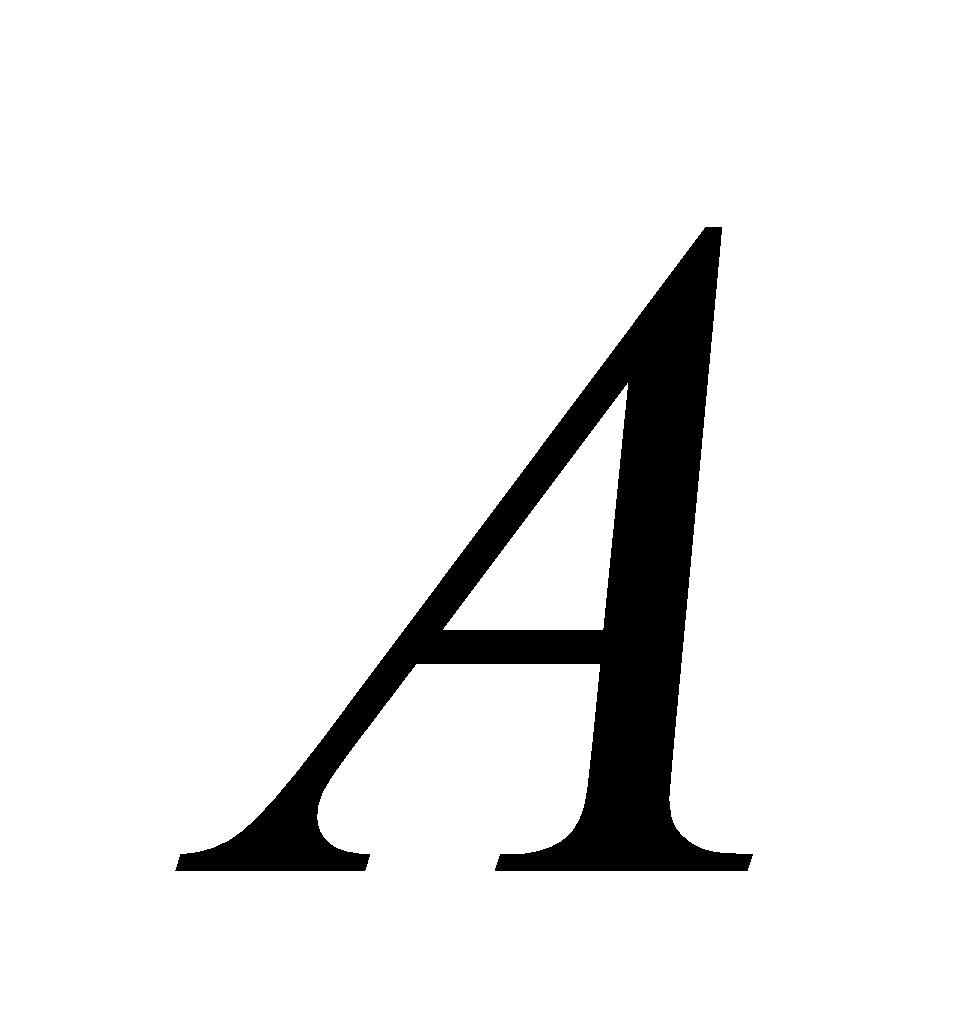and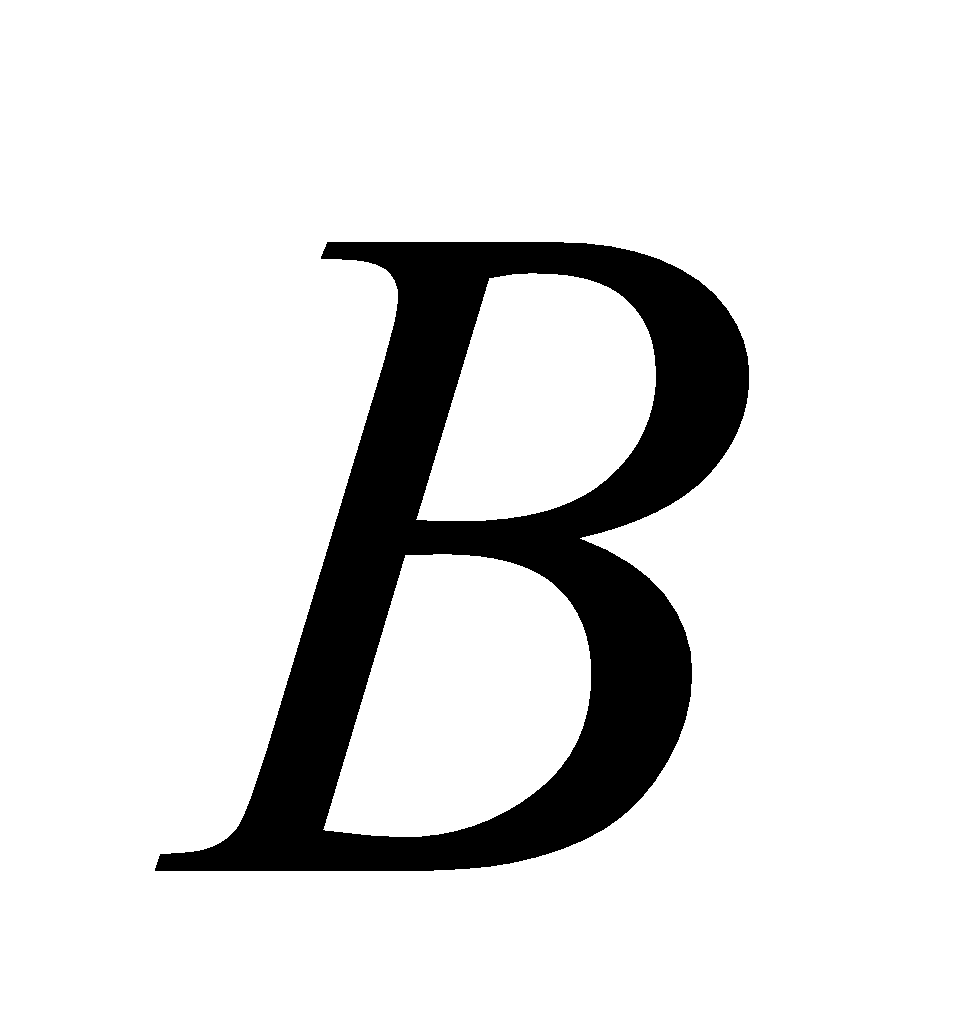are two matrices such that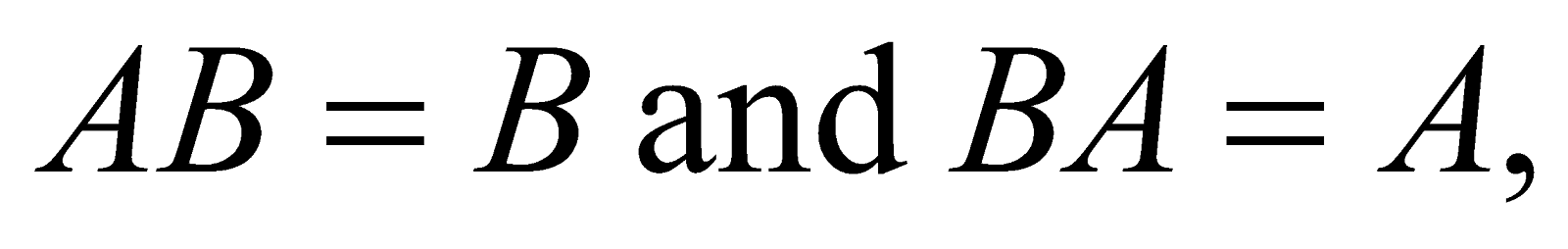then find the value of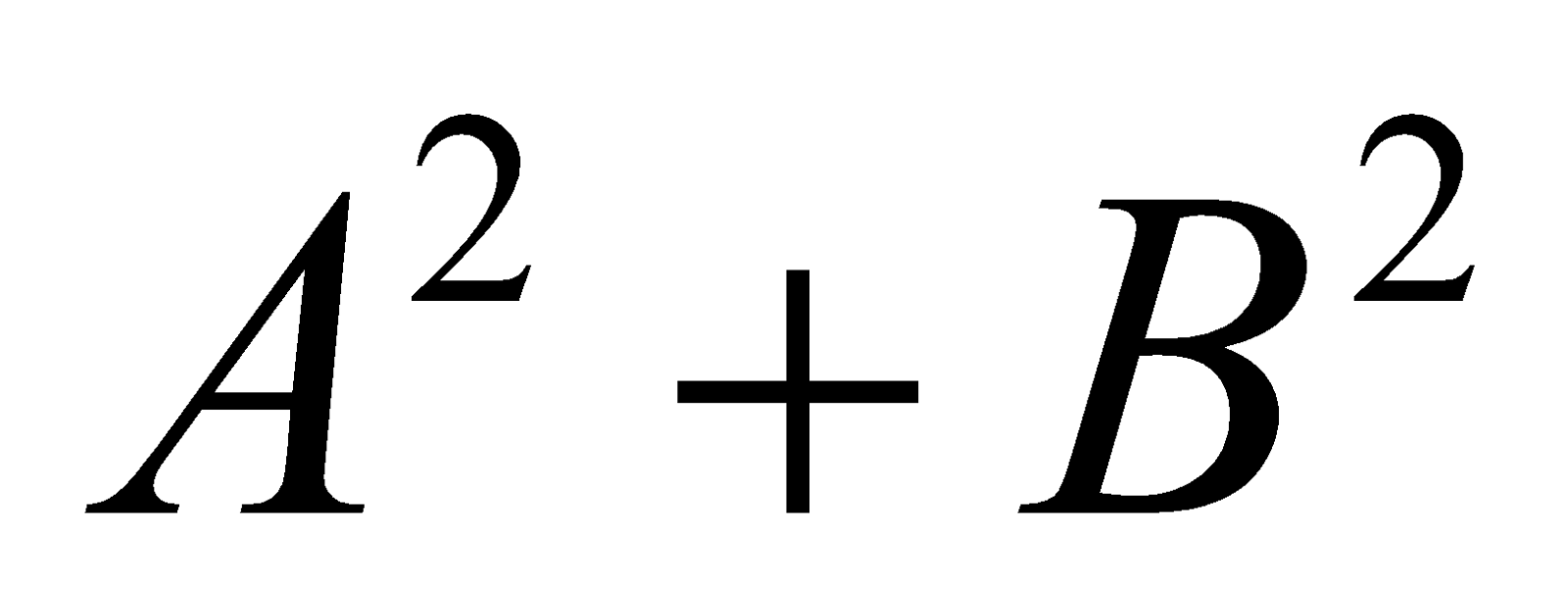.
2. Show that the diagonal elements of a skew-symmetric matrix are all zero.
5. Prove that the function given by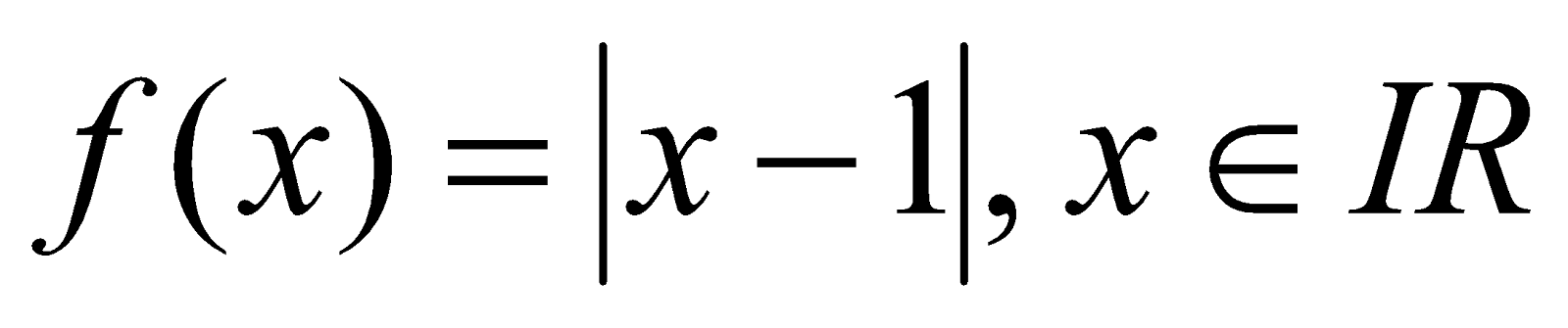is not differentiable at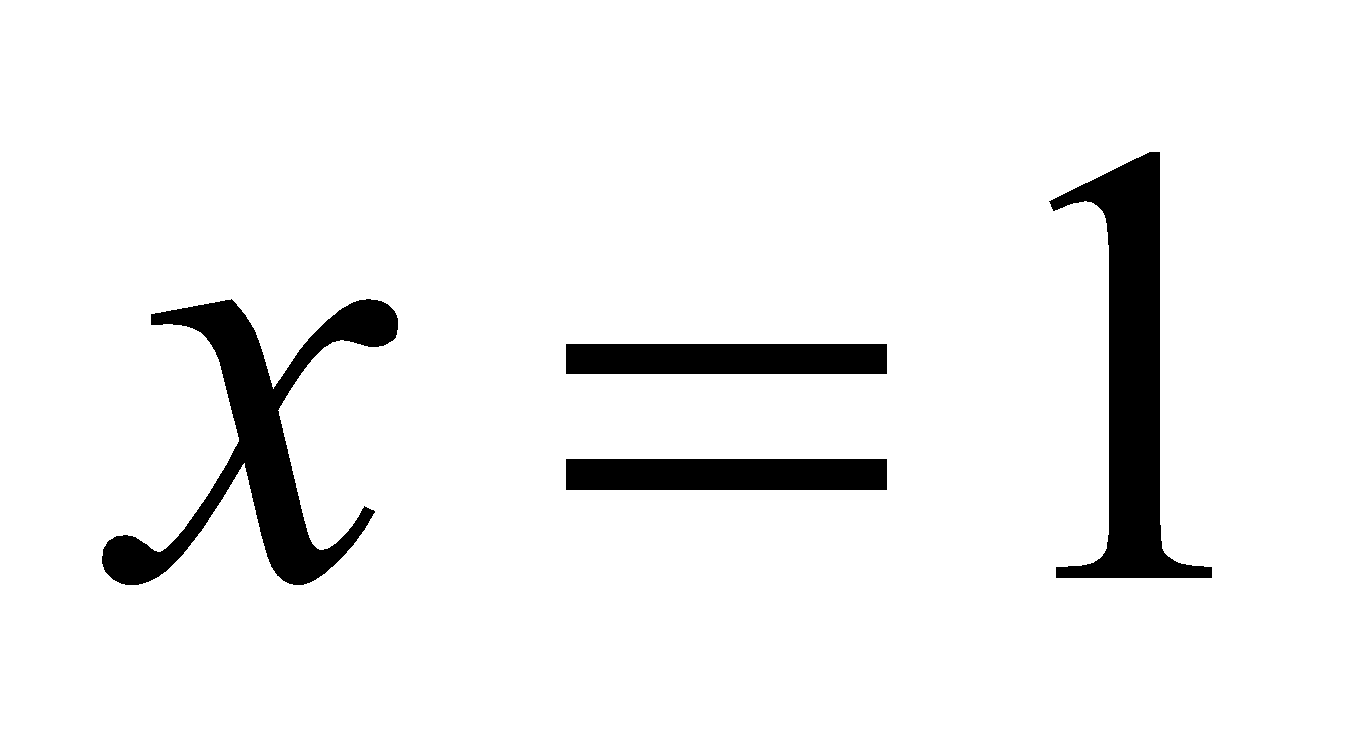. Also examine the continuity at that point. 4
Or
If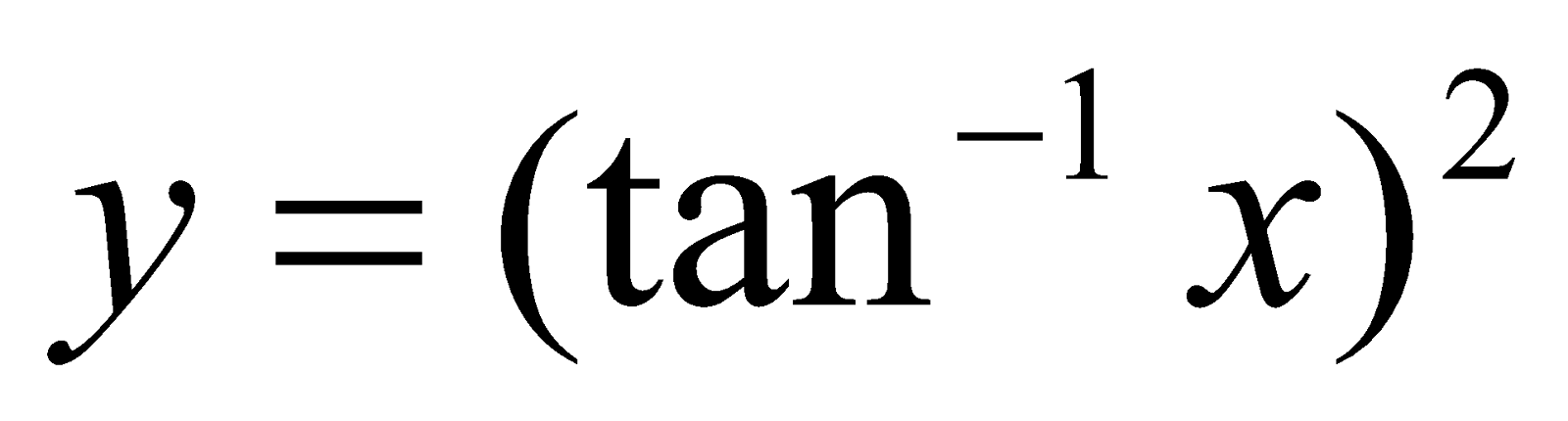show that 4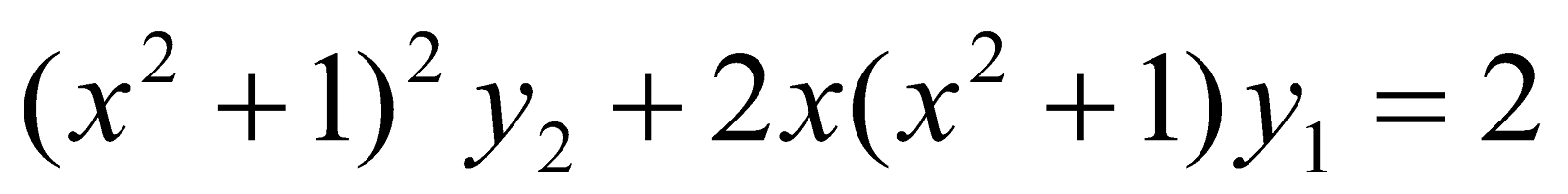6. If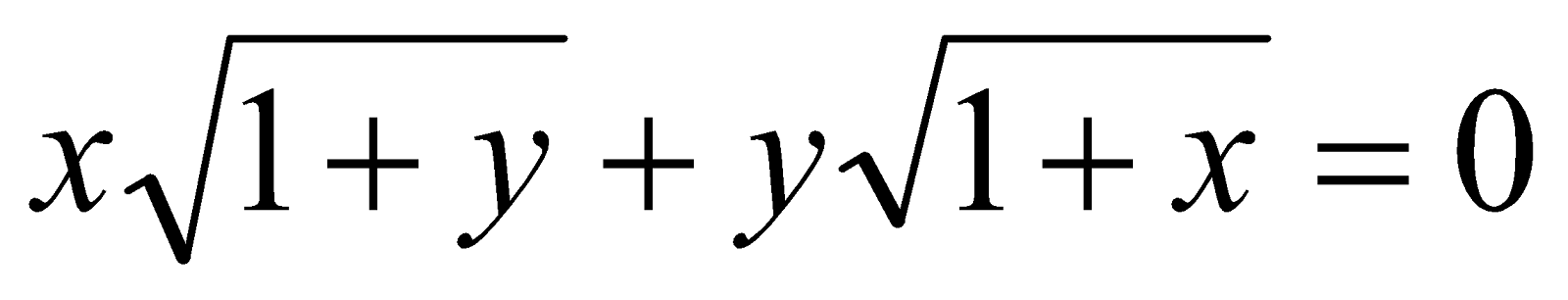then show that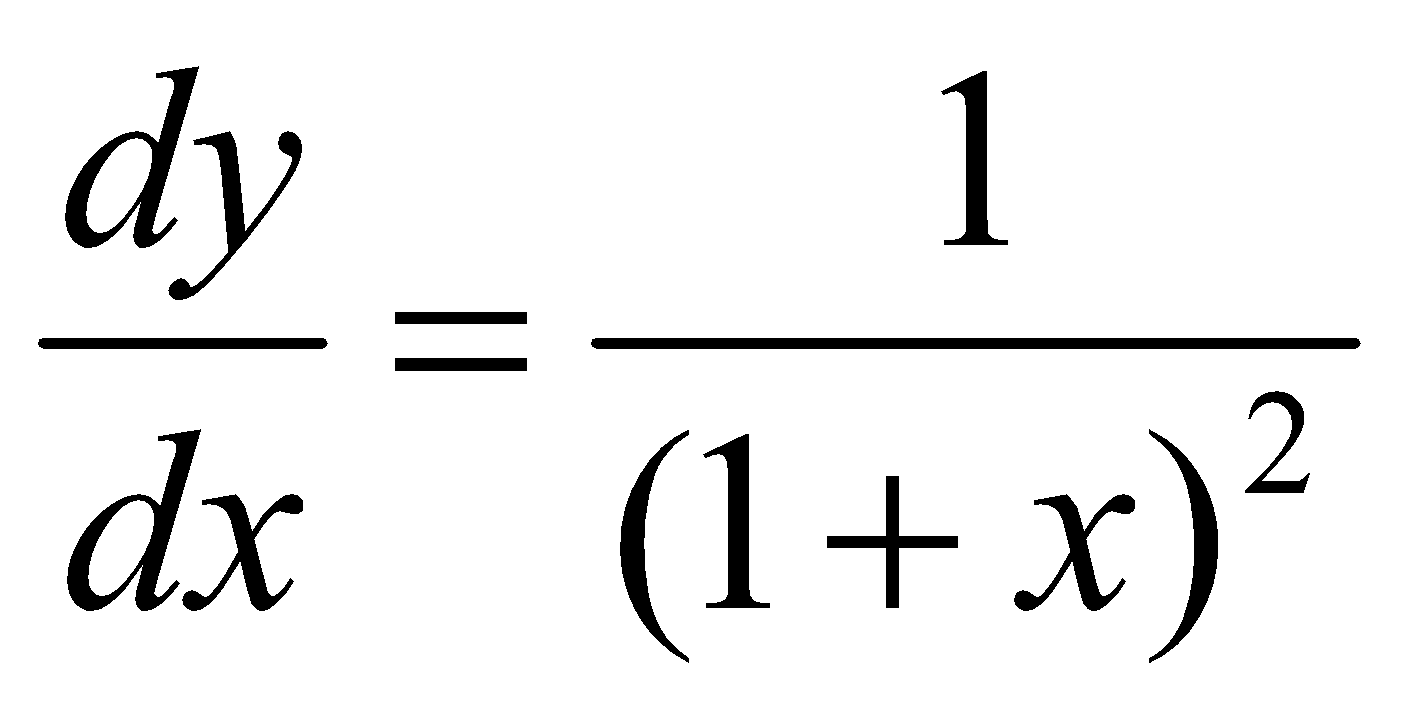. 4
7. Integrate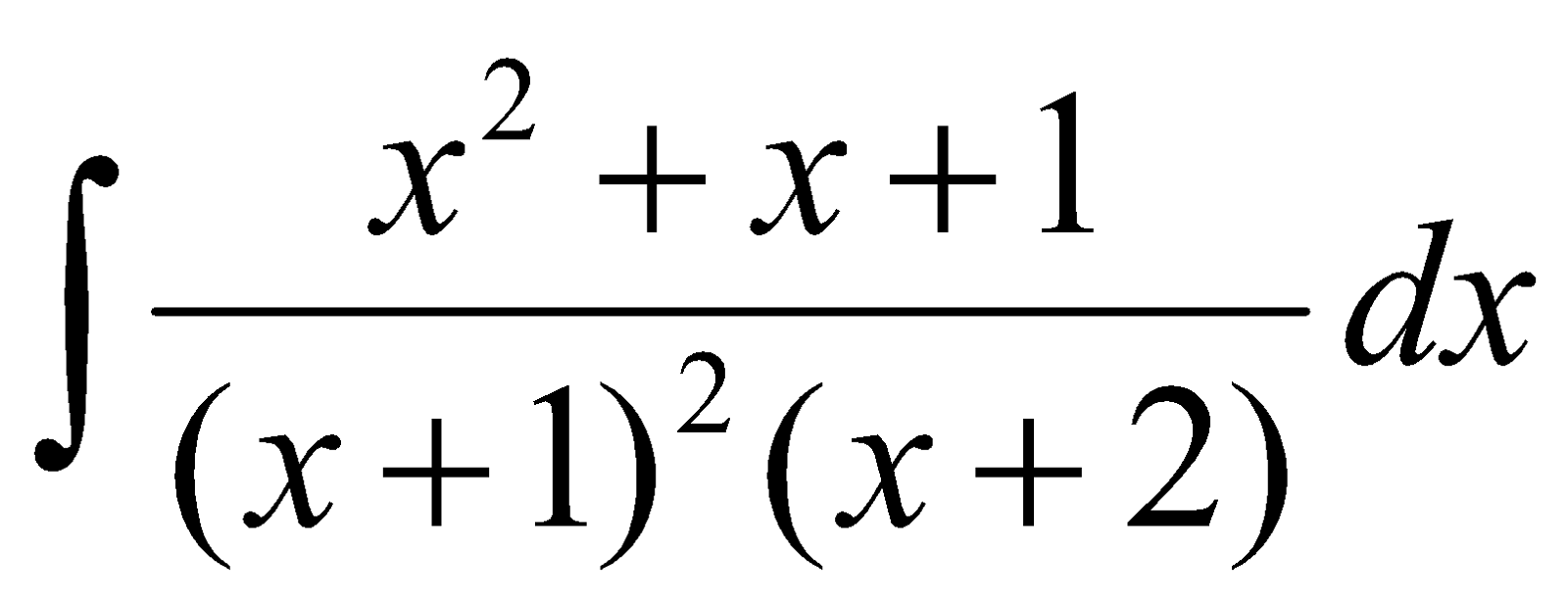8. EvaluateOr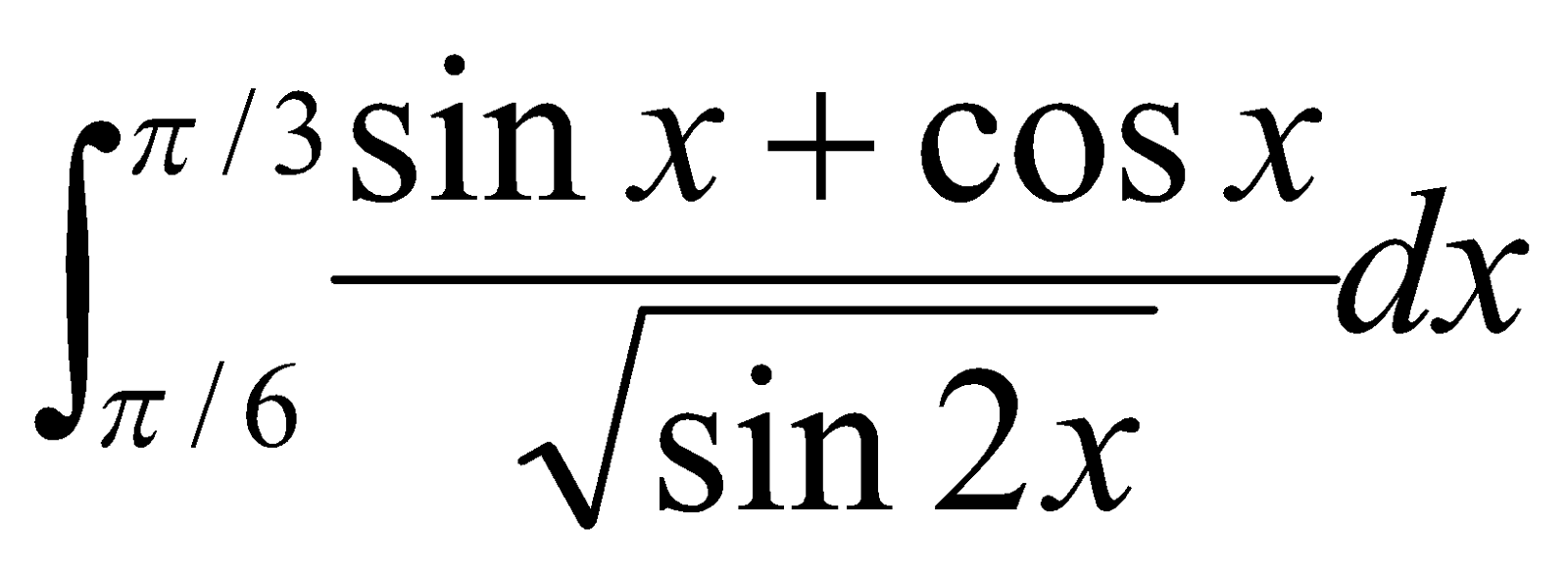9. Answer any two of the following questions: 4x2=8
1. Solve2. Find the particular solution of the differential equation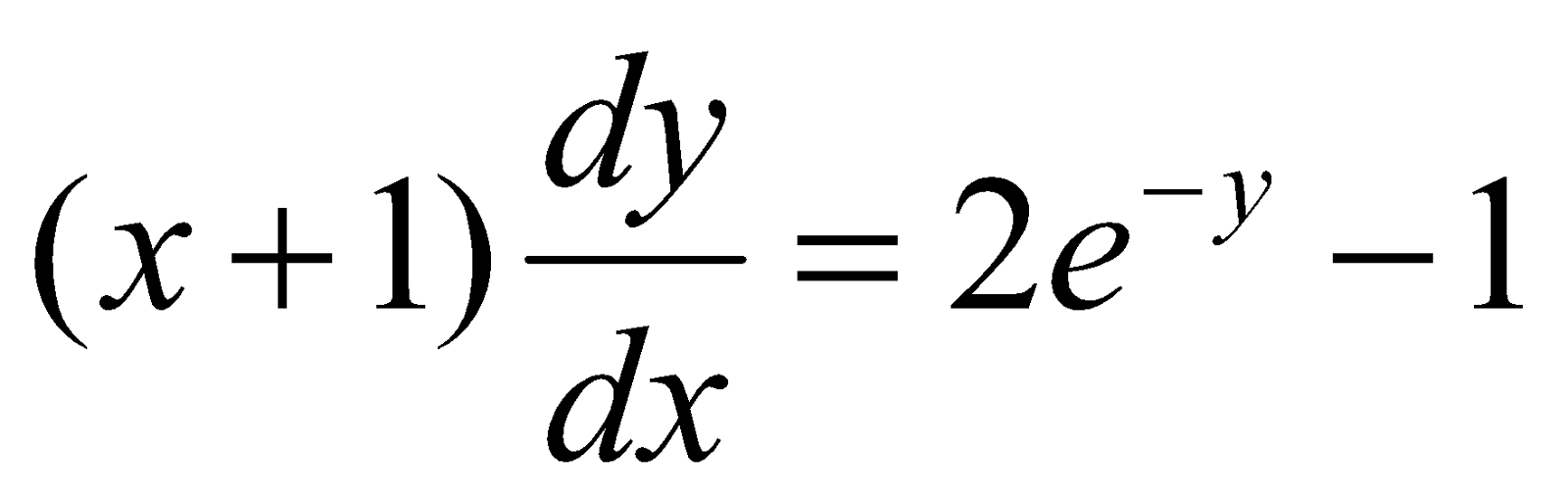given that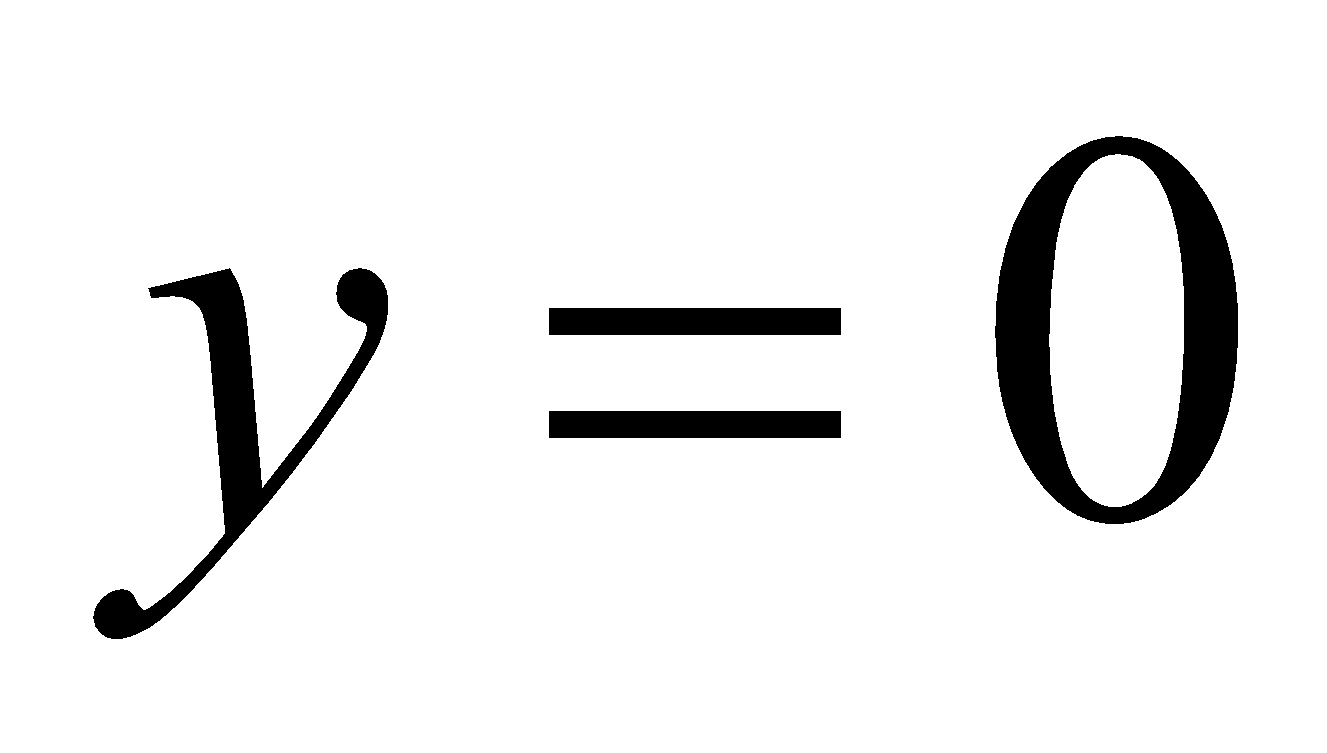when.
3. Find the solution curve passing through the point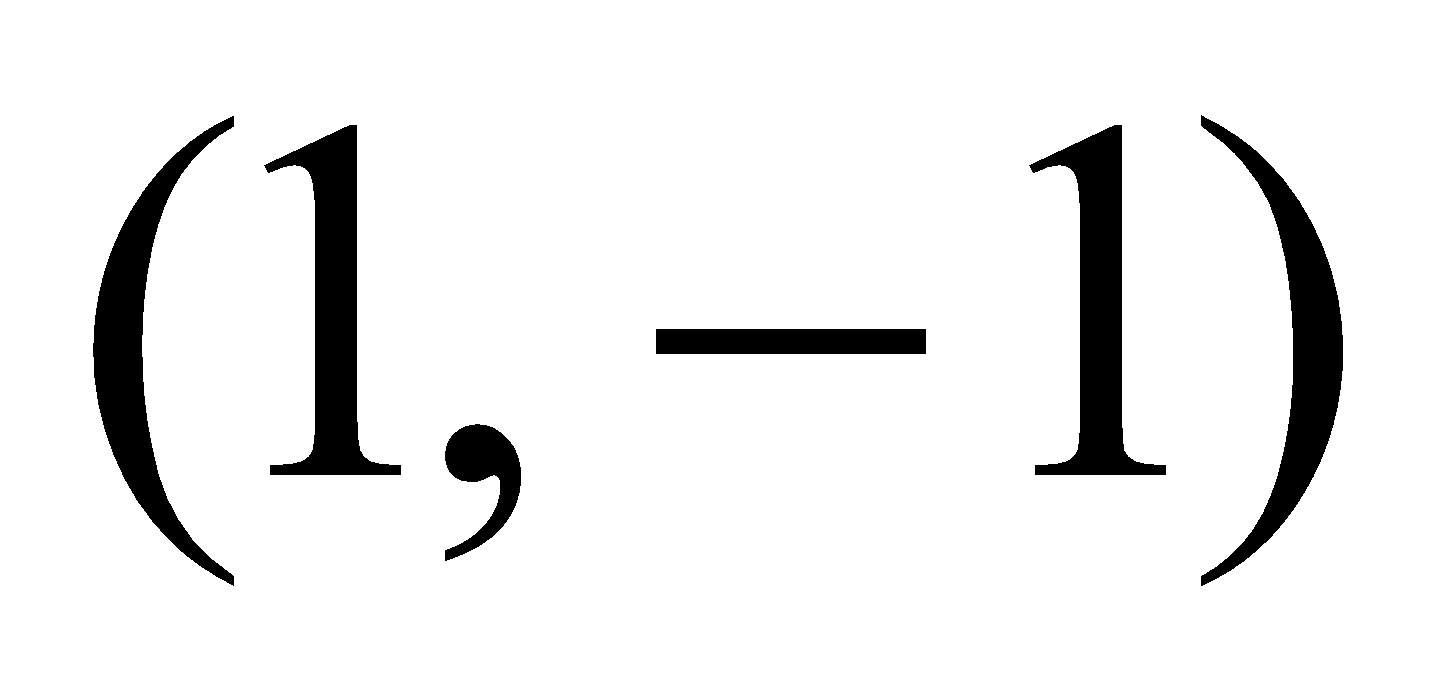of the differential equation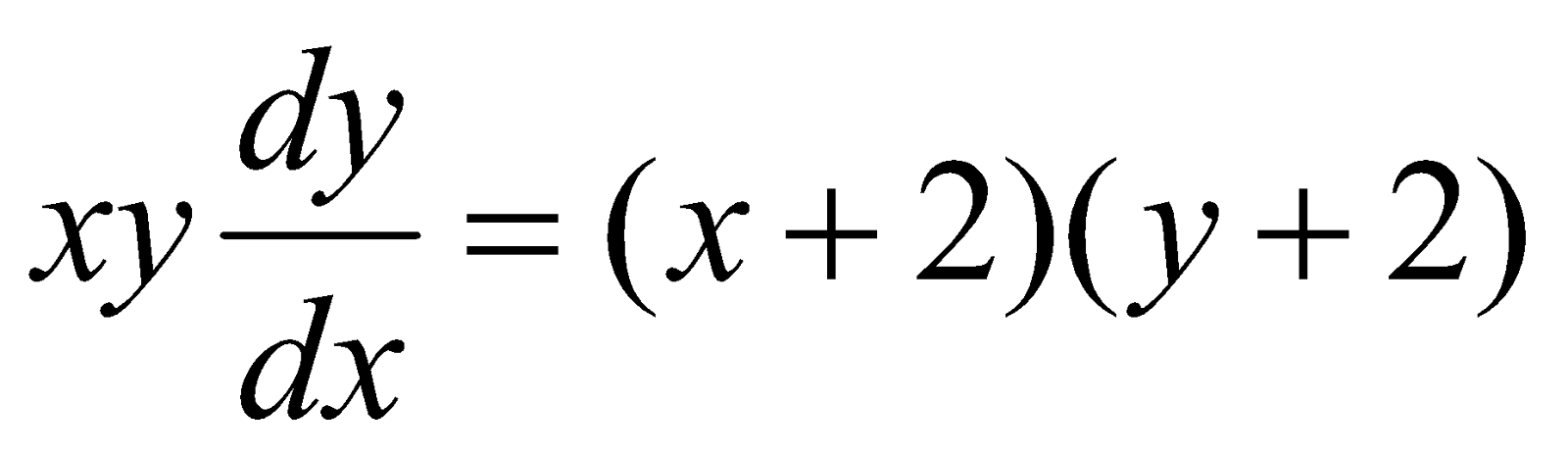10. Show that, for any vector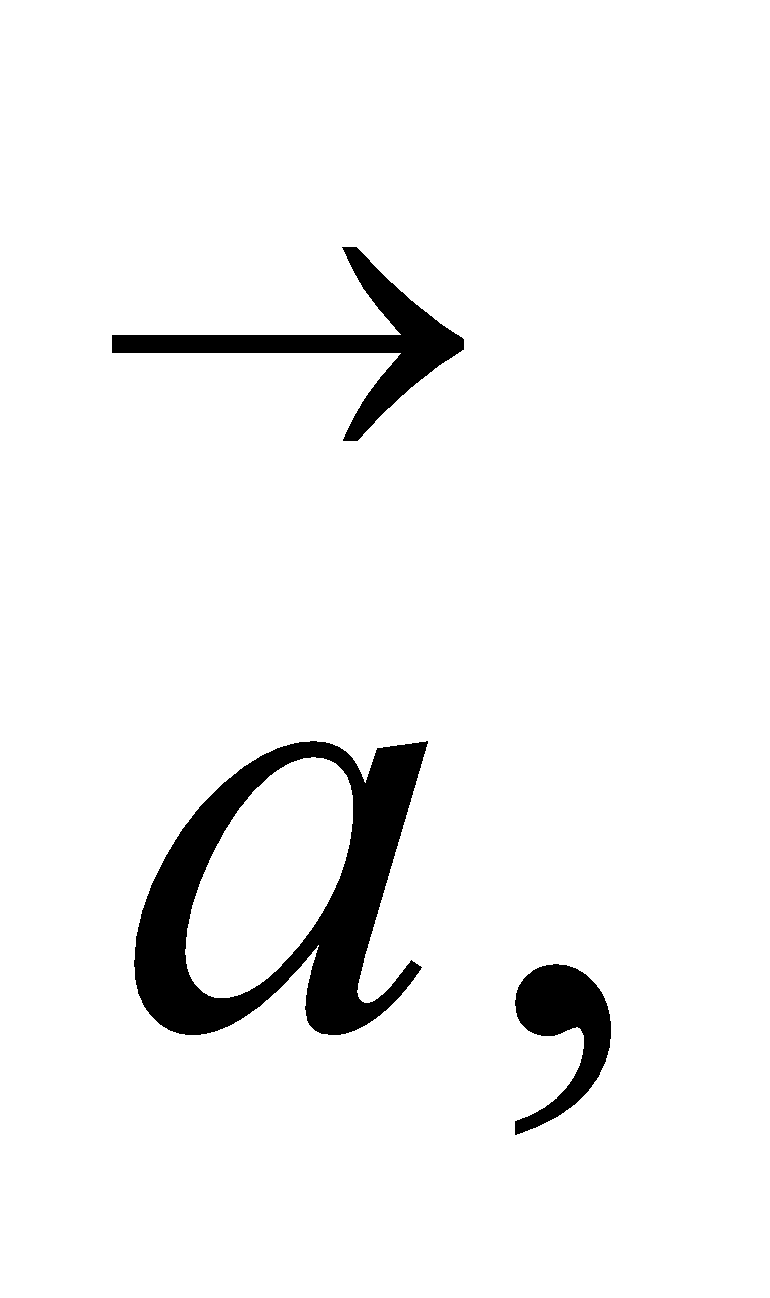4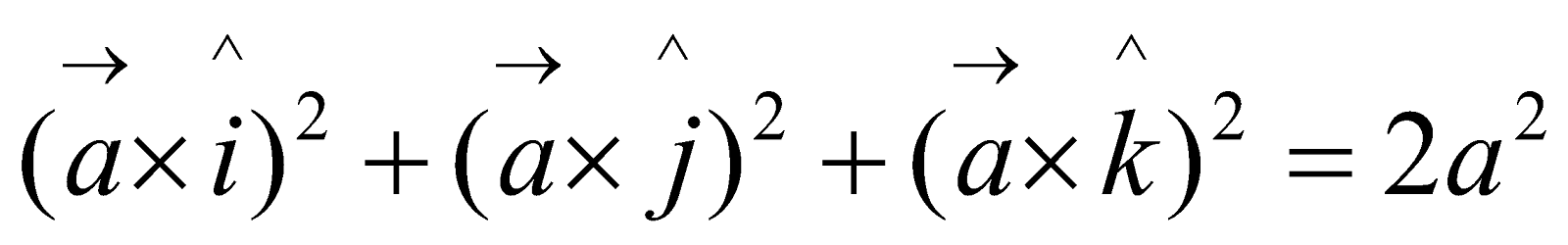Or
Let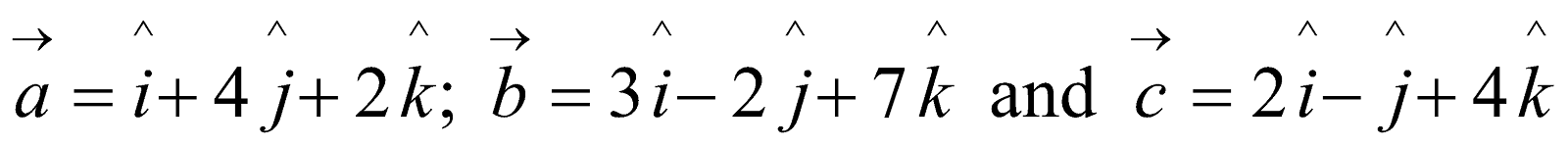. Find the vectorwhich is perpendicular to both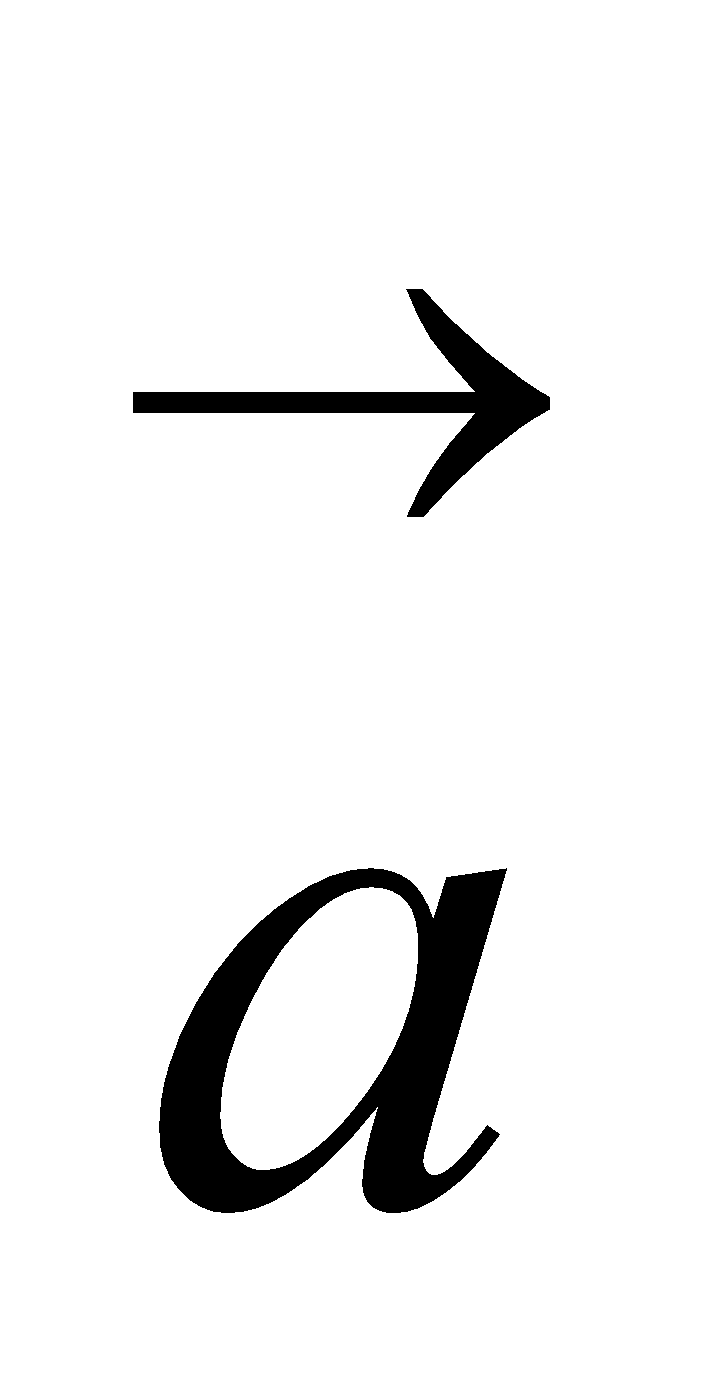,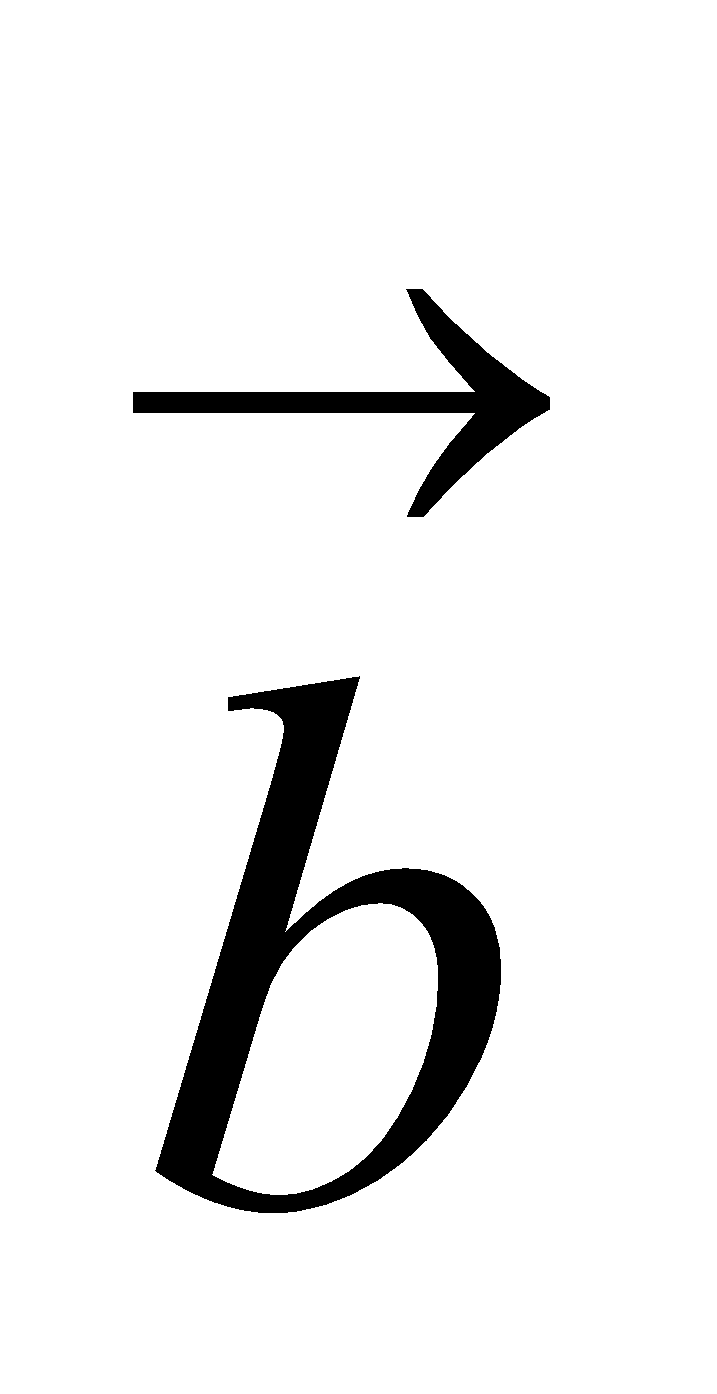and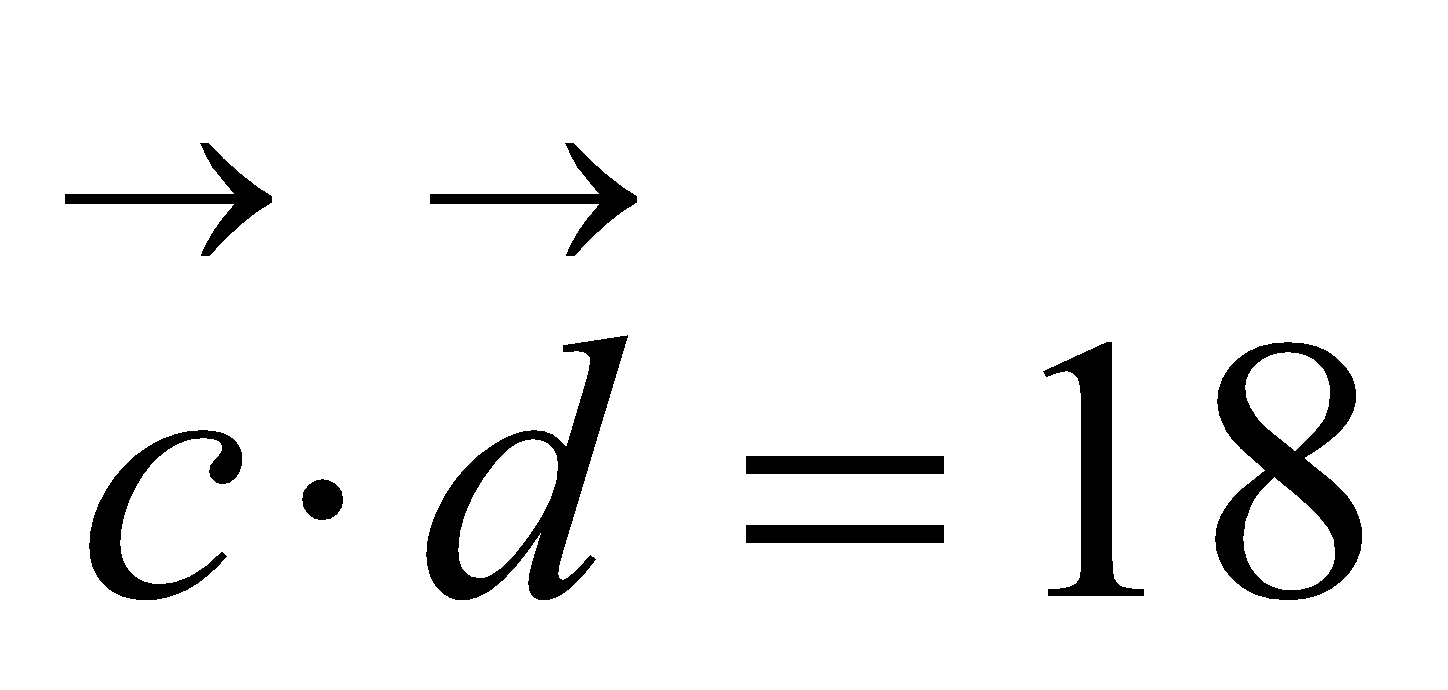. 4
11. Find the co-ordinates of the foot of the perpendicular drawn from origin to the plane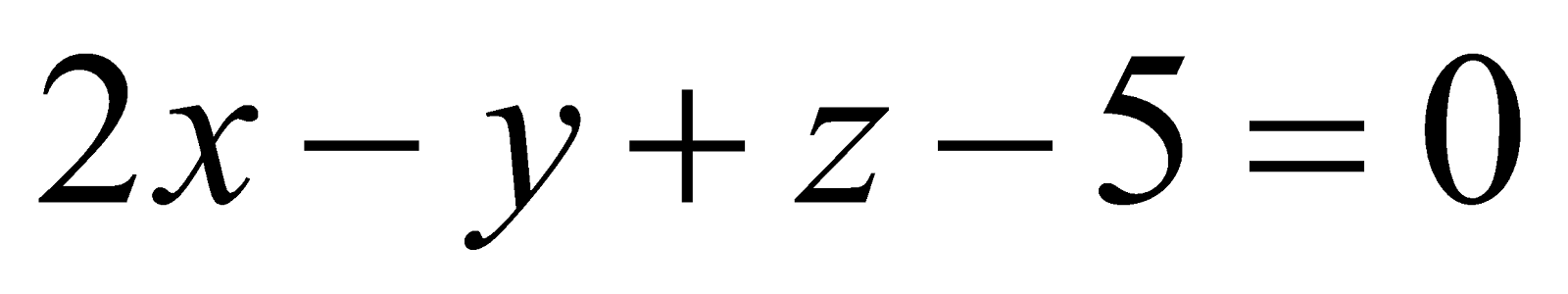.     4
12. Letdenote the sum of the numbers obtained when two fair dice are rolled. Find the variance of.     4
Or
Two cards are drawn simultaneously (or successively without replacement) from a well shuffled pack of 52 cards. Find the mean and variance of the number of kings. 4
13. If a, b, c is real numbers and 6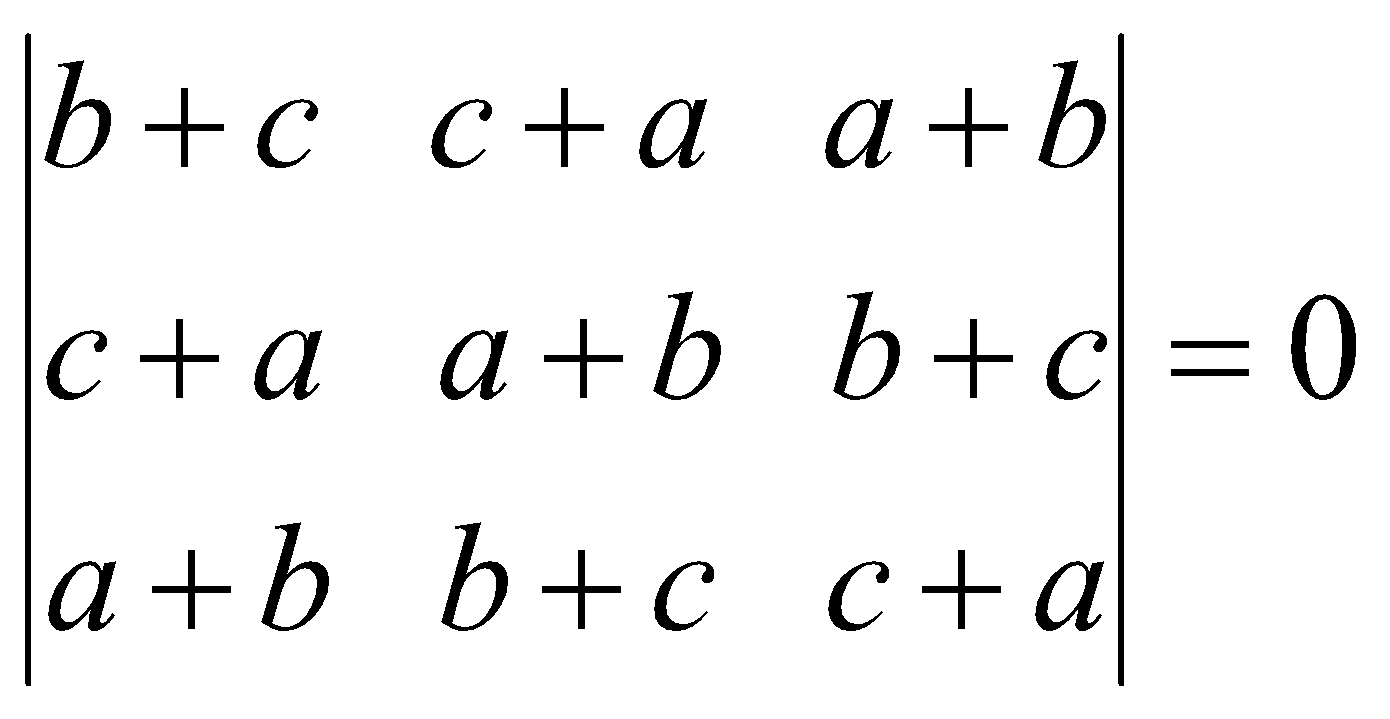Prove that either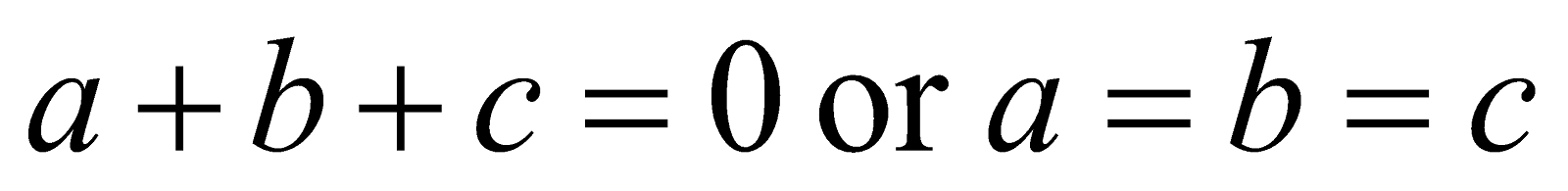Or
Without expanding at any stage prove that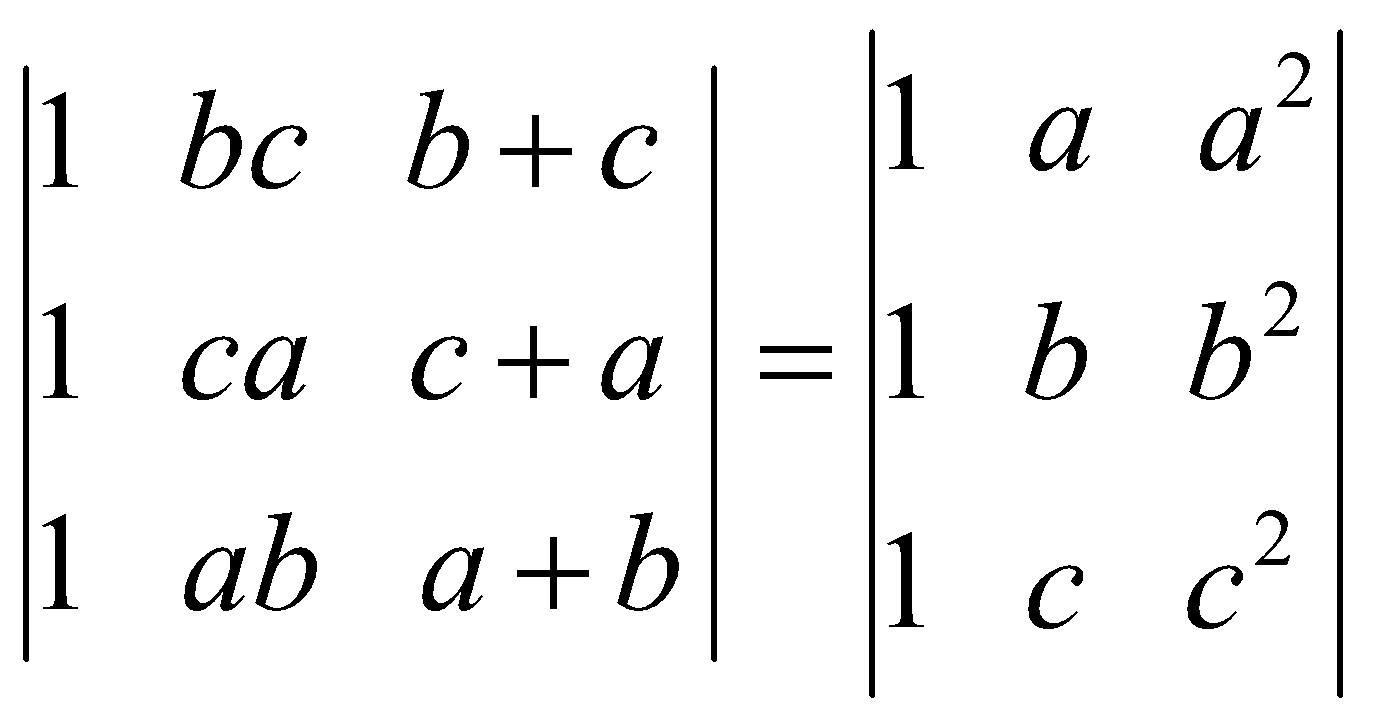14. Find the equation of tangents of the curve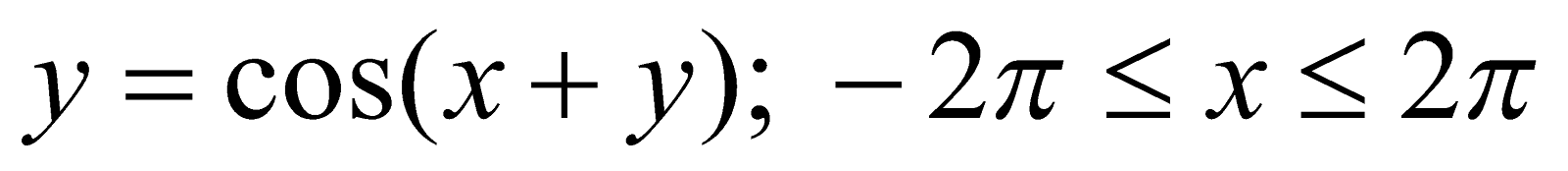that are parallel to the line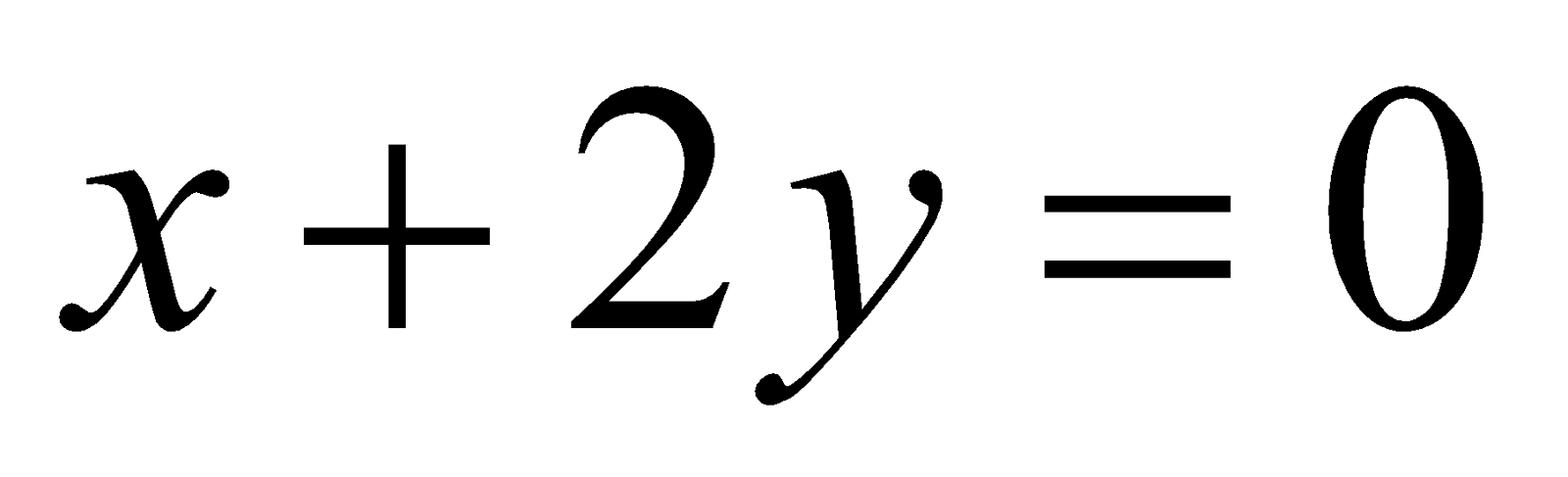. 6
Or
Show that 3+3=6
1. The functiongiven by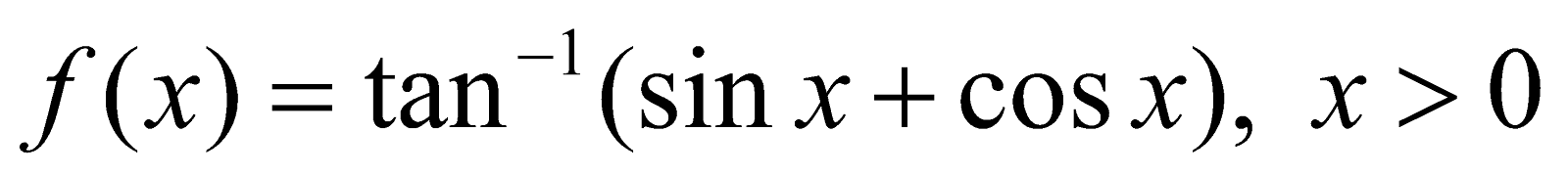Is always a strictly increasing function in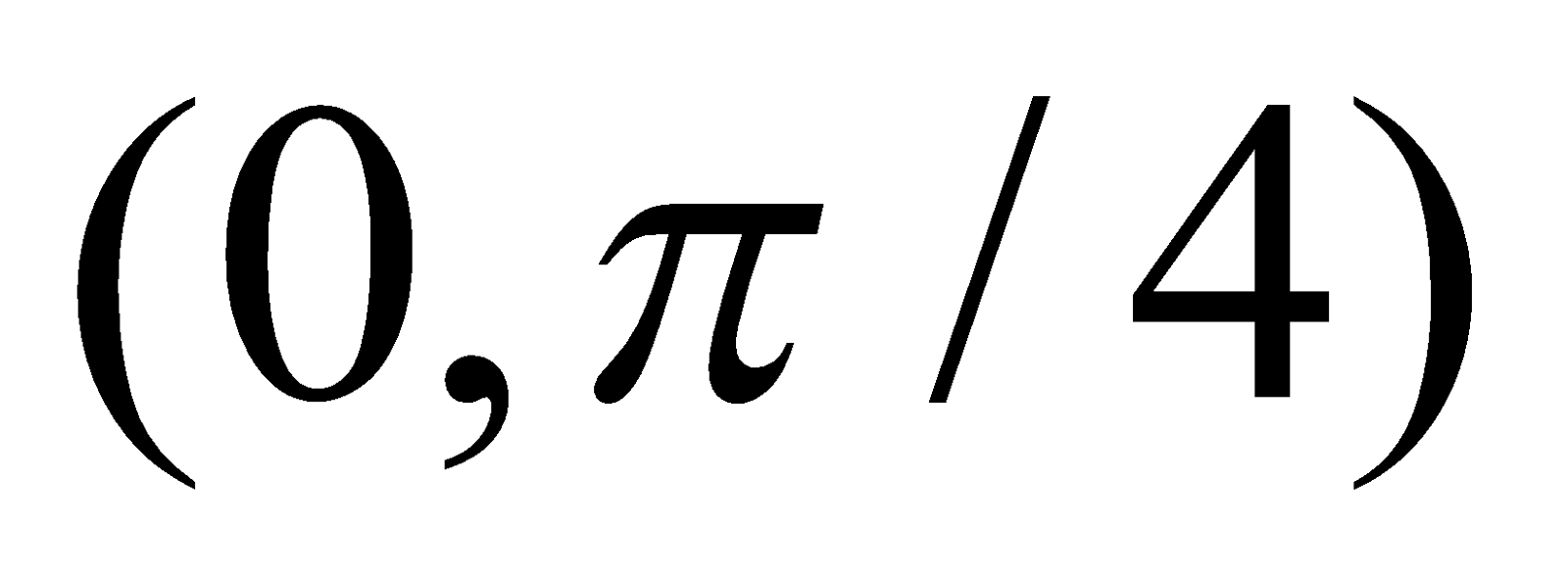.
1.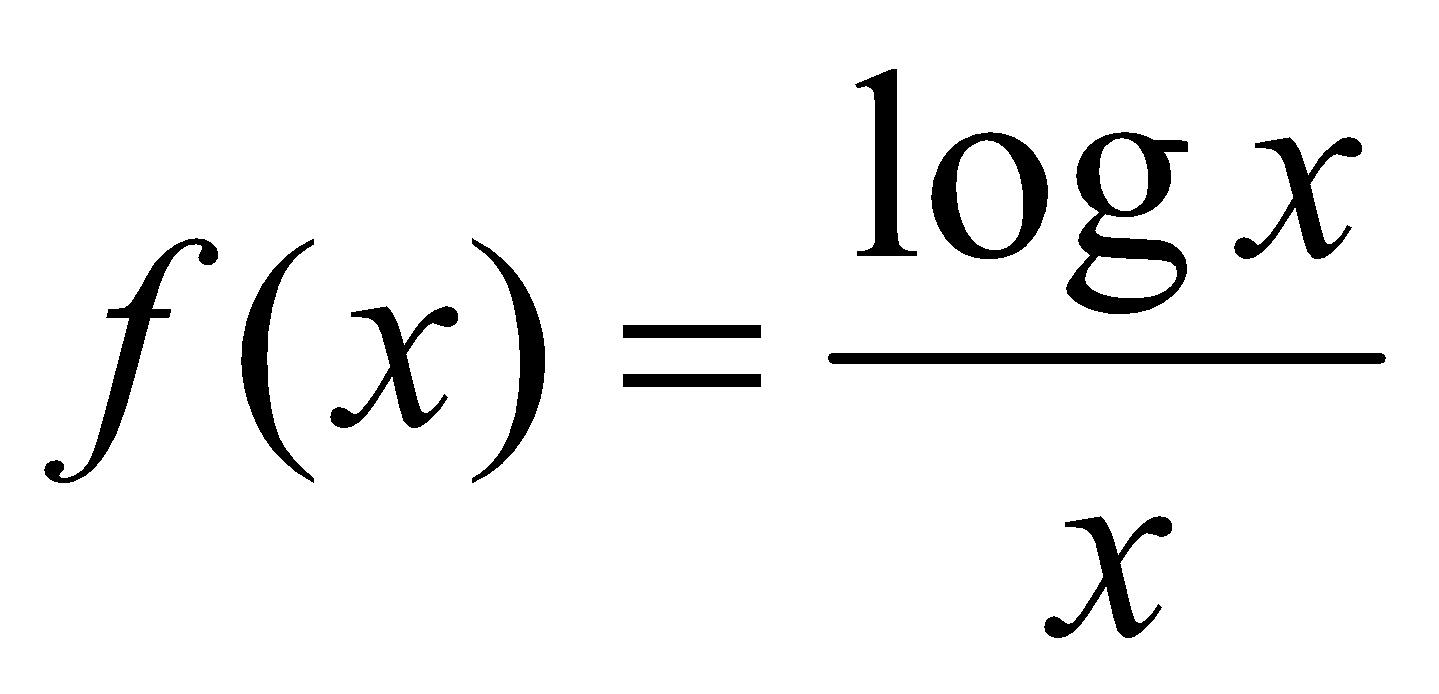Has maximum at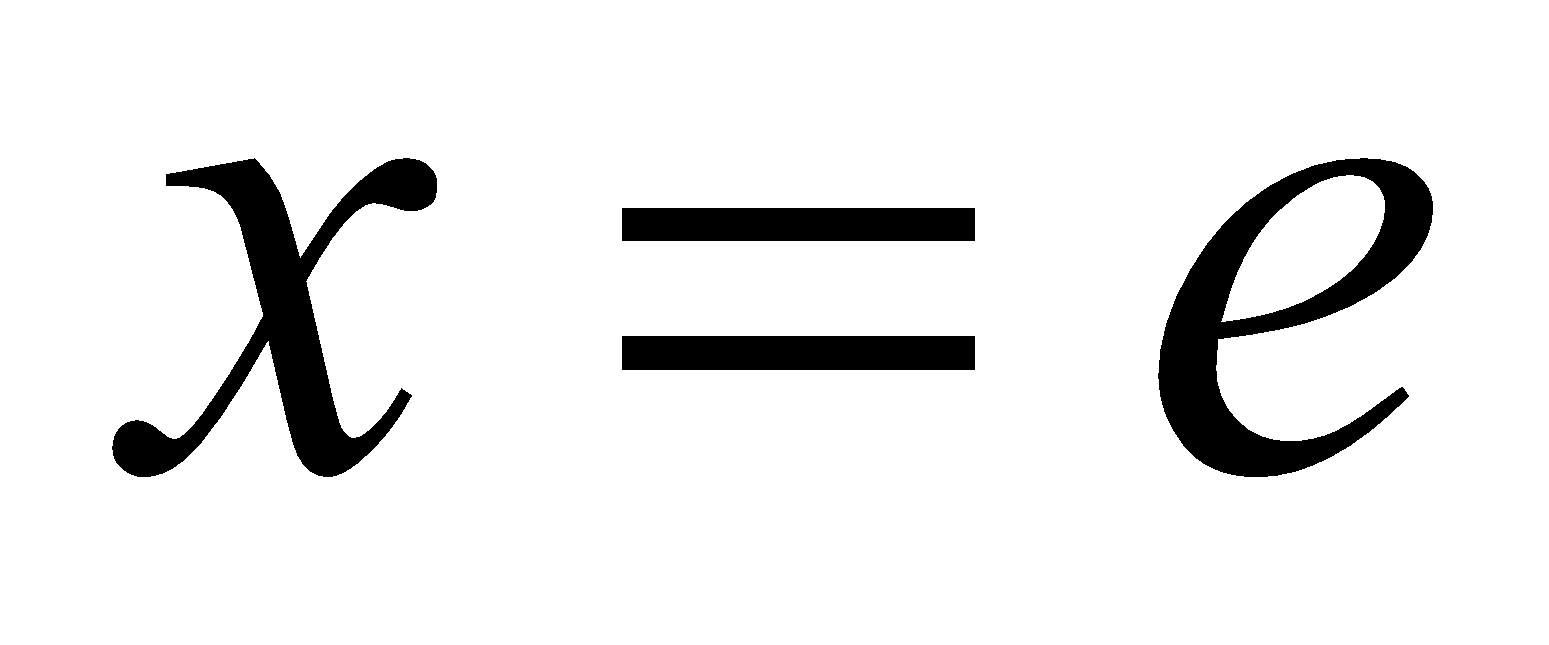.
15. Evaluate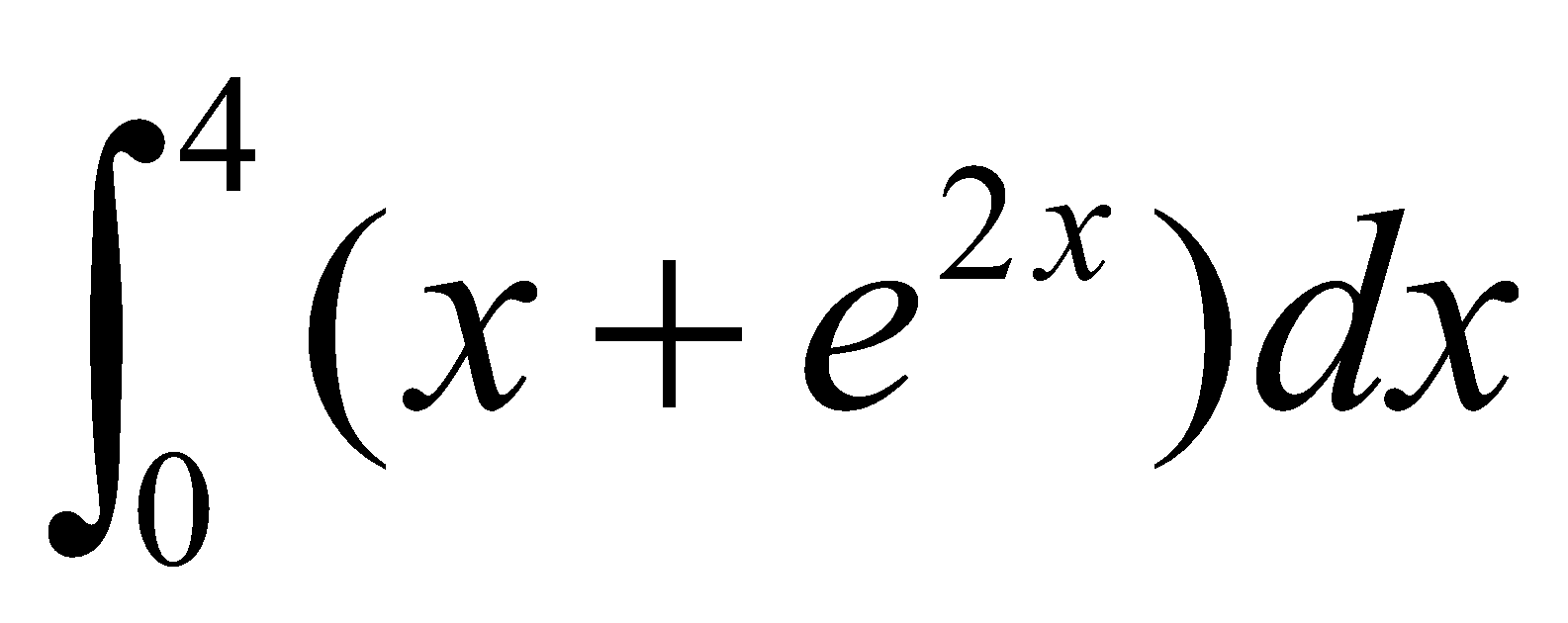by expressing it as the limit of a sum.
16. Find the angle between the line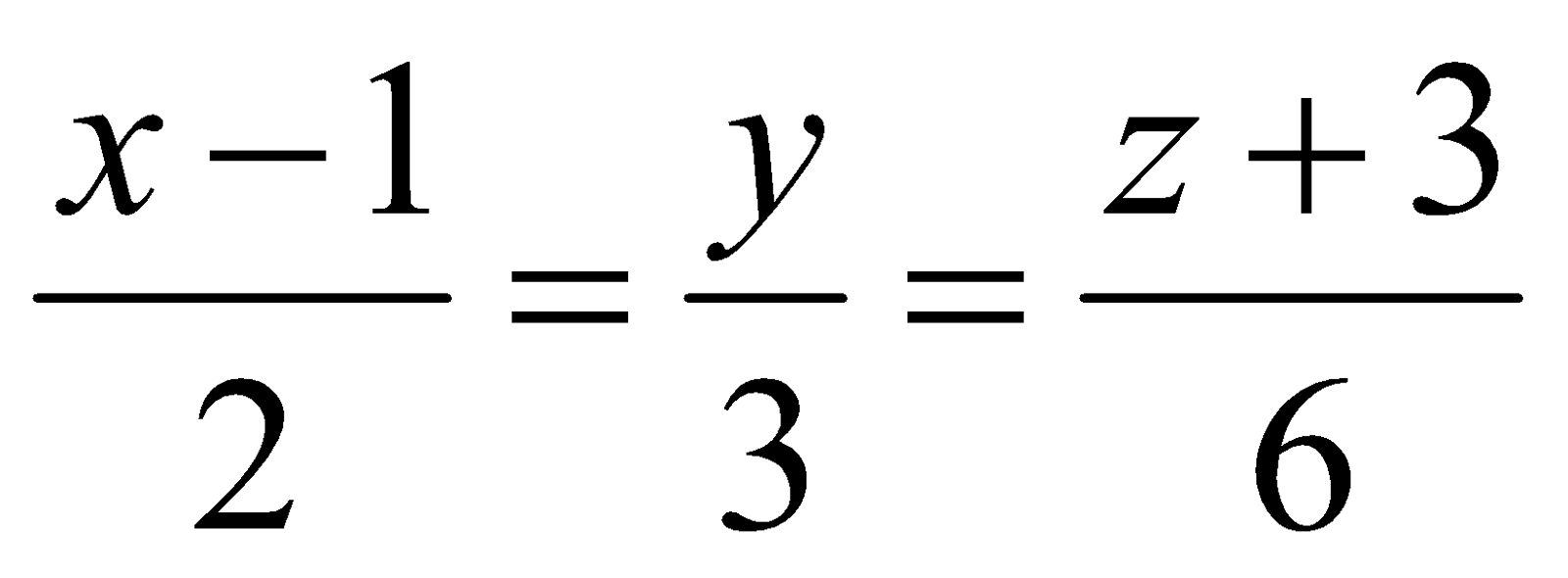and the plane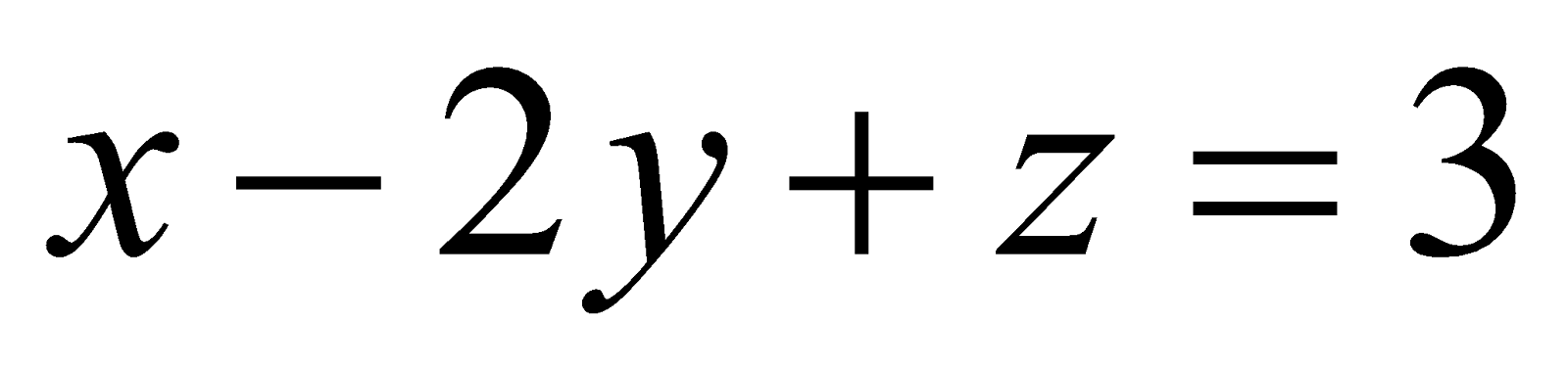. Also find the point at which the line intersects the given plane. 6
Or
Find the shortest distance and the equation of shortest distance between the lines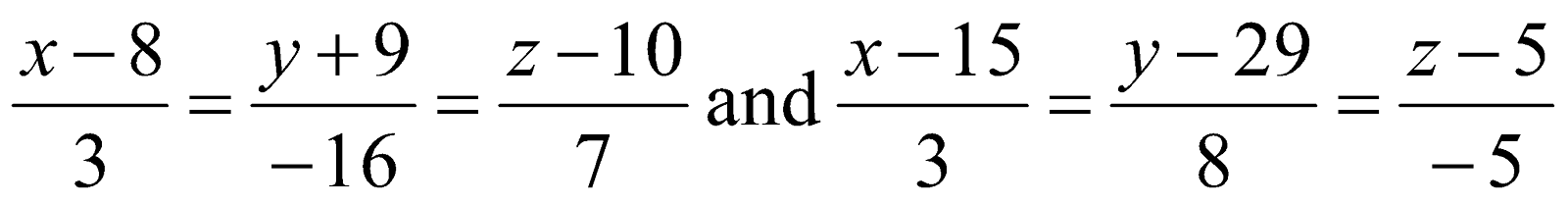.
17. Find the area of the region in the 1st quadrant enclosed by the x-axis, the line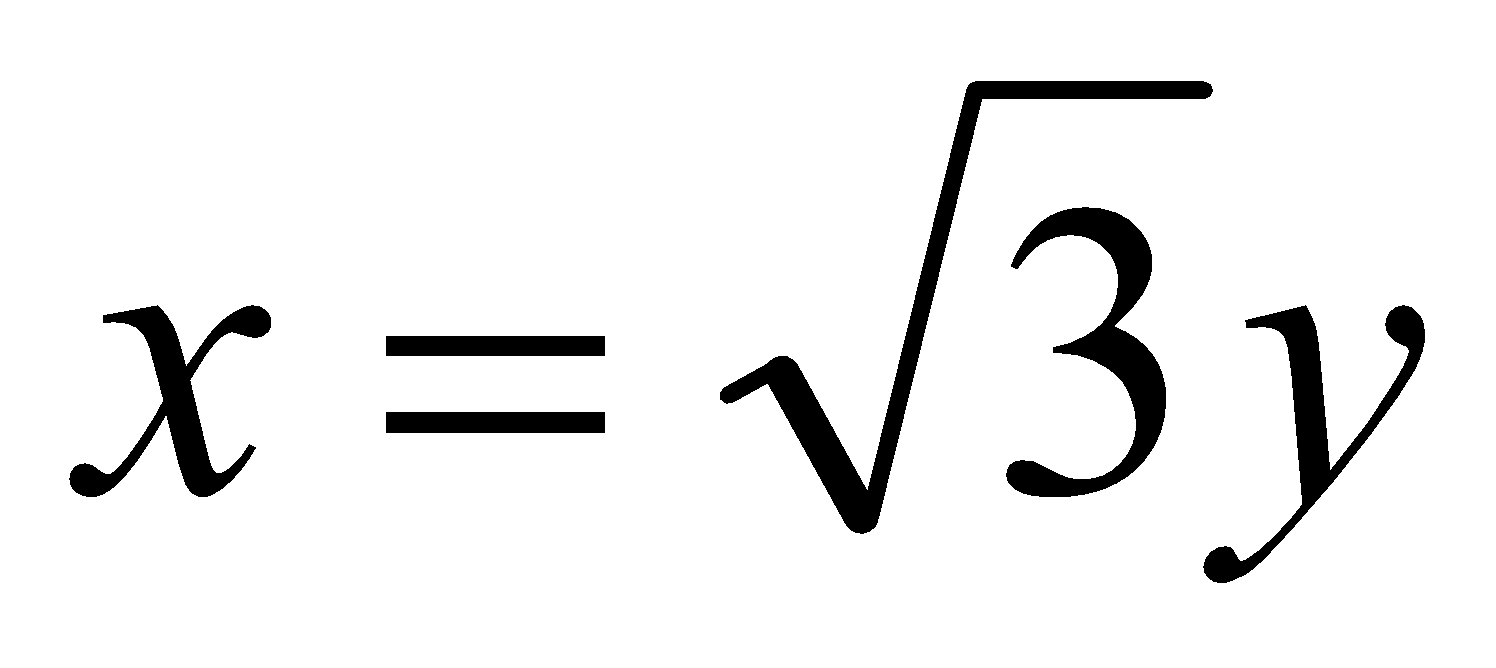and the circle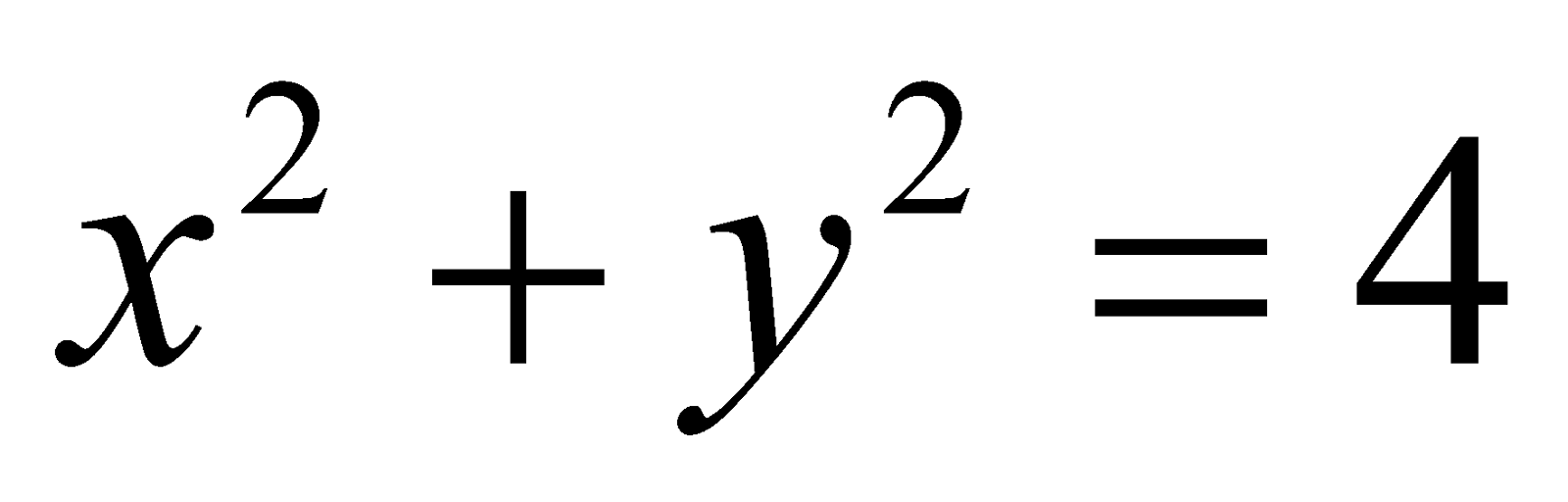6
Or
Find the area of the region bounded by the parabola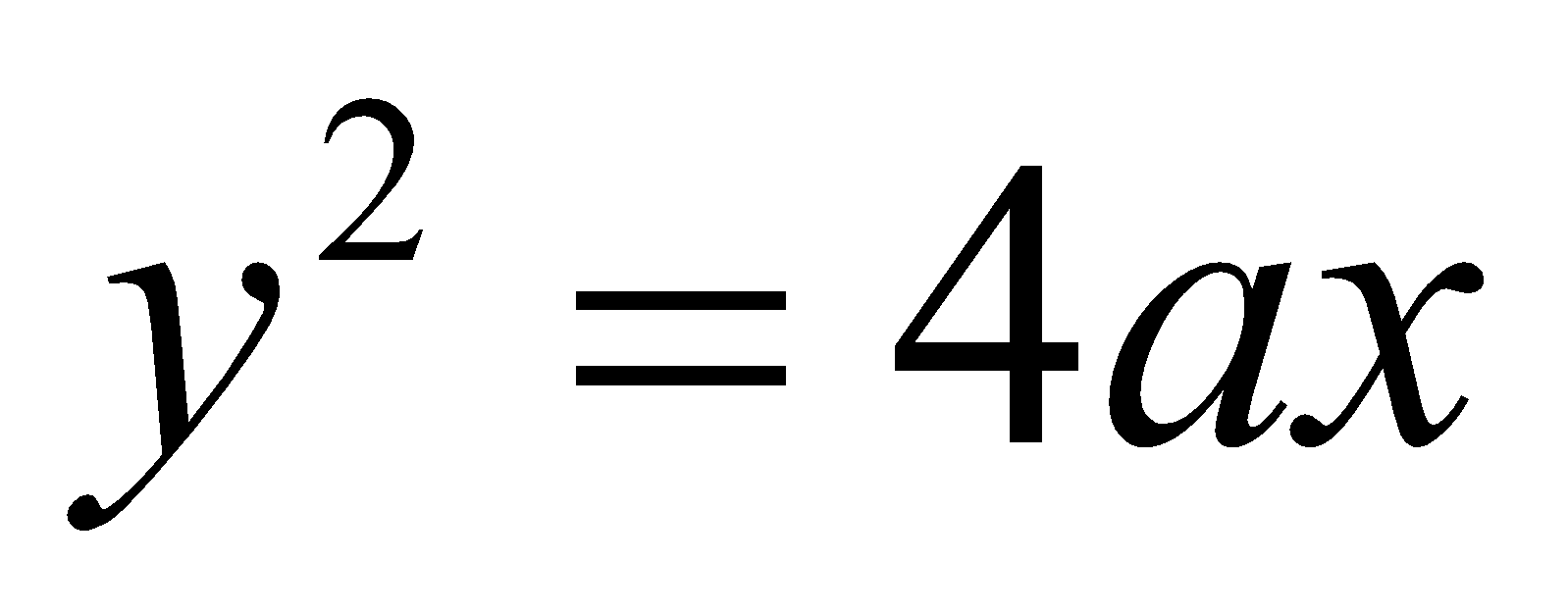and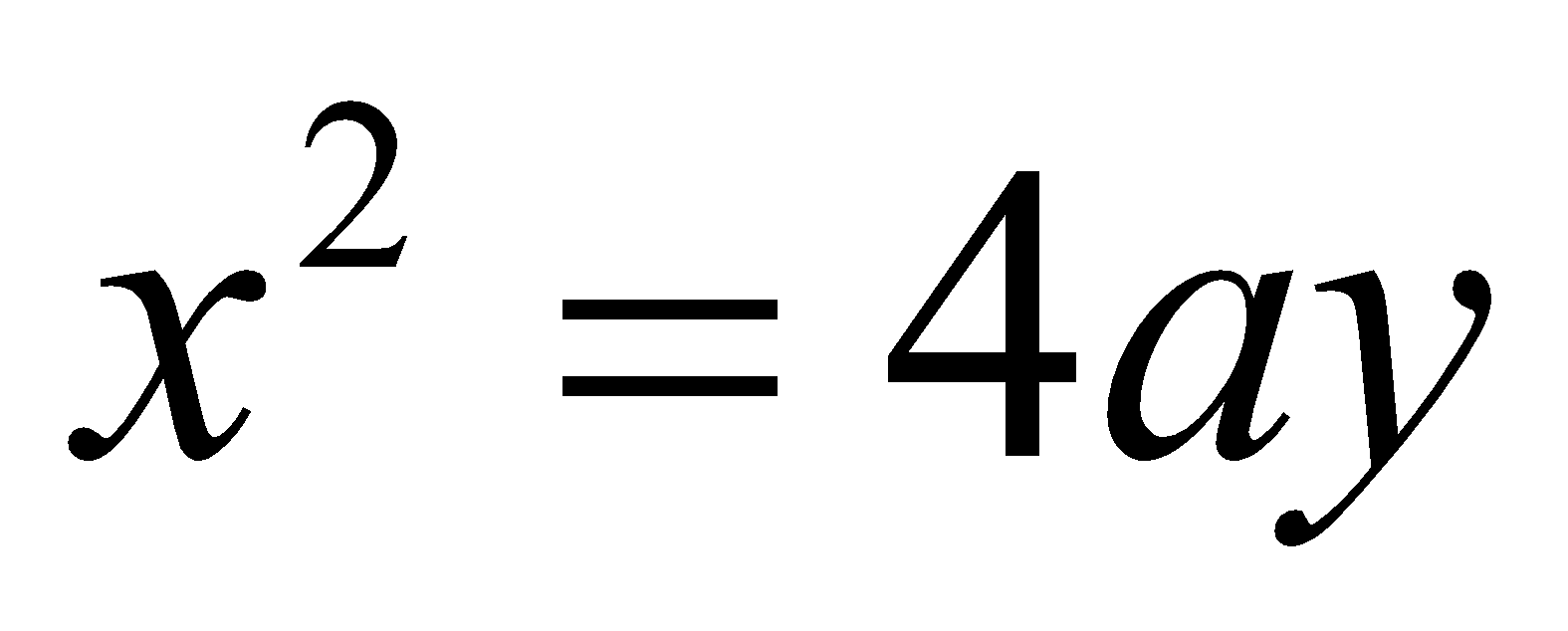. 6
18. Showing the feasible region, solve graphically the following linear programming problem: 6
Maximize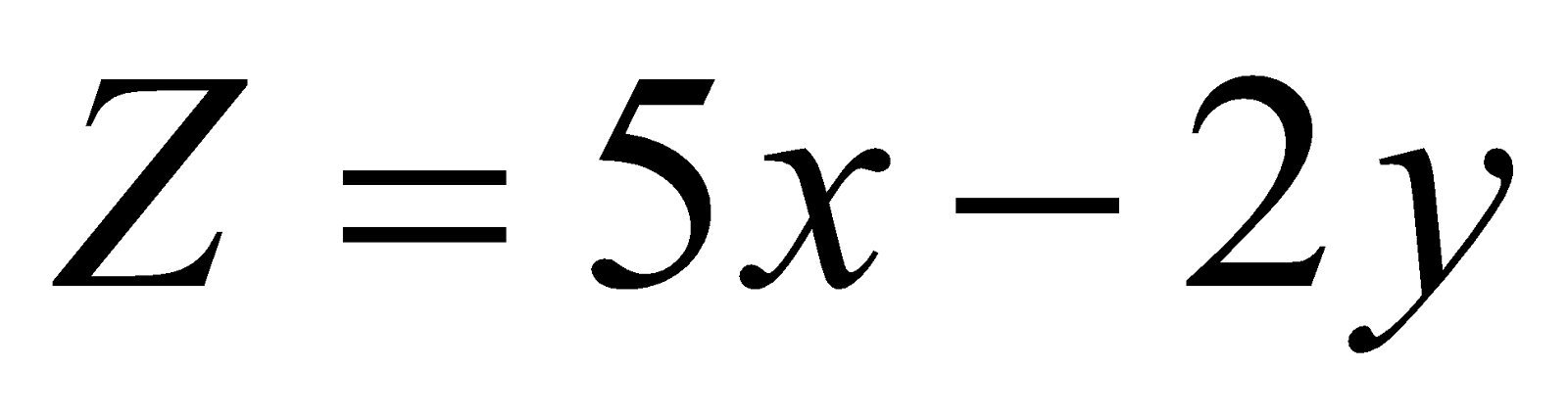Subject to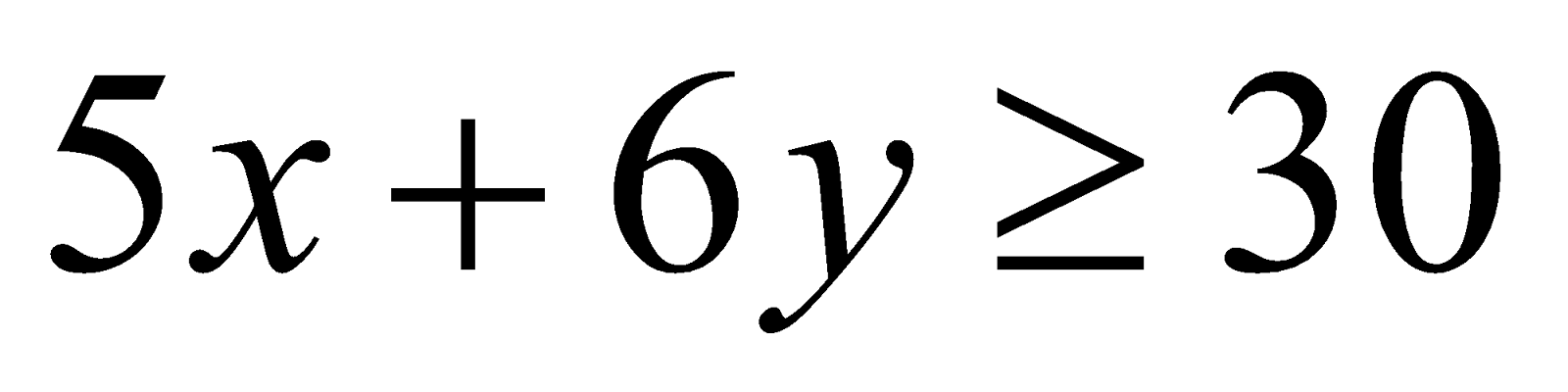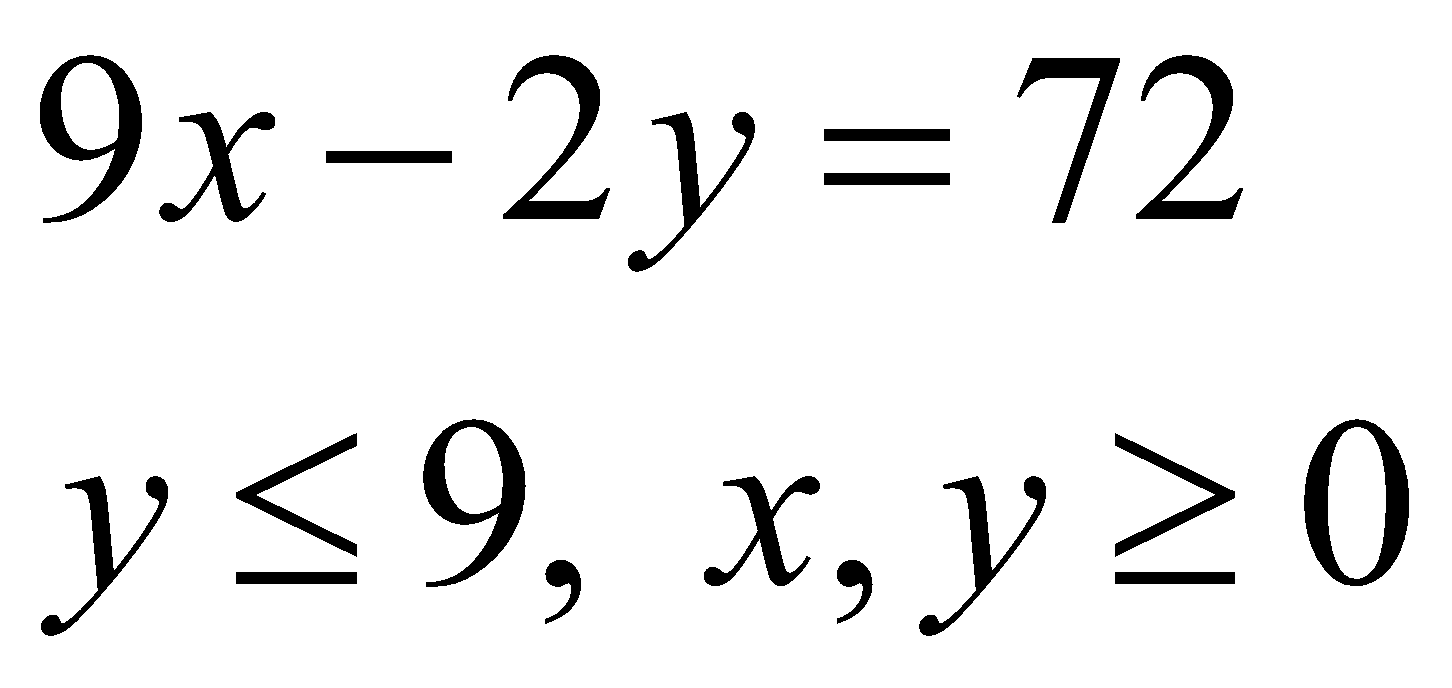19. There are three coins. One is a two headed coin (having heads on both sides), another is a biased coin that turns up heads 75% of the time and third is an unbiased coin. One of the three coins is chosen at random and tossed. It shows head. What is the probability that it was the two headed coin? 6
Or
Probability of solving a specific problem independently by A and B are 1/2 and 1/3 respectively. If both try to solve the problem independently, find the probability that

1. The problem is solved.
2. Exactly one of them solves the problem. 3+3=6